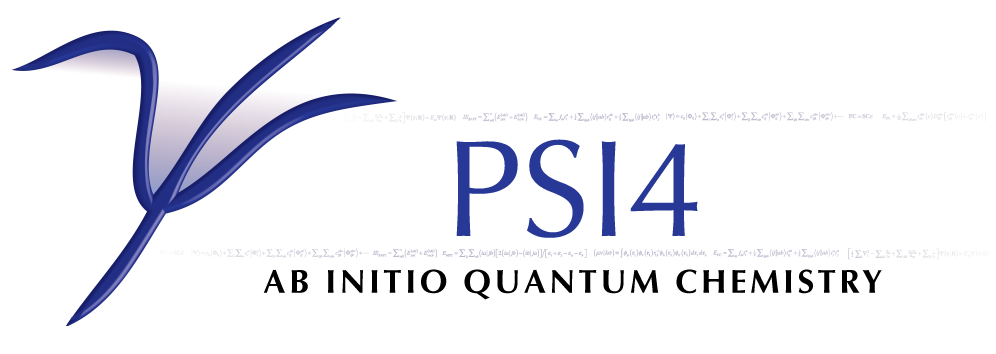# Keywords by Alpha¶

A_RAS3_MAX (DETCI)

DETCI — maximum number of alpha electrons in RAS III

• Type: integer
• Default: -1
AA_M_FILE (TRANSQT)
• Type: integer
• Default: PSIF_MO_AA_TEI
AB_M_FILE (TRANSQT)
• Type: integer
• Default: PSIF_MO_AB_TEI
ABCD (CCENERGY)
• Type: string
• Possible Values: NEW, OLD
• Default: NEW
ABCD (CCEOM)
• Type: string
• Possible Values: NEW, OLD
• Default: NEW
ABCD (CCLAMBDA)
• Type: string
• Default: NEW
ABCD (CCRESPONSE)
• Type: string
• Default: NEW
ACTIVE (DETCI)

DETCI — An array giving the number of active orbitals (occupied plus unoccupied) per irrep (shorthand to make MCSCF easier to specify than using RAS keywords)

• Type: array
• Default: No Default
ACTIVE (PSIMRCC)

PSIMRCC — The number of active orbitals per irrep

• Type: array
• Default: No Default

OPTKING — Do add bond coordinates at nearby atoms for non-bonded systems?

AEL (CCDENSITY)

CCDENSITY (Expert) — Do compute the approximate excitation level? See Stanton and Bartlett, JCP, 98, 1993, 7034.

AIO_CPHF (SAPT)

SAPT — Do use asynchronous disk I/O in the solution of the CPHF equations? Use may speed up the computation slightly at the cost of spawning an additional thread.

AIO_DF_INTS (SAPT)

SAPT — Do use asynchronous disk I/O in the formation of the DF integrals? Use may speed up the computation slightly at the cost of spawning an additional thread.

ALGORITHM (DCFT)

DCFT — The algorithm to use for the density cumulant and orbital updates in the energy computation. Two-step algorithm (default) is generally more efficient and shows better convergence than simultaneous

• Type: string
• Possible Values: TWOSTEP, SIMULTANEOUS
• Default: TWOSTEP
ANALYZE (CCENERGY)

CCENERGY — Do ?

ANALYZE (CCRESPONSE)

CCRESPONSE — Do ?

AO_BASIS (CCDENSITY)

CCDENSITY — The algorithm to use for the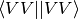terms

• Type: string
• Possible Values: NONE, DISK, DIRECT
• Default: NONE
AO_BASIS (CCENERGY)

CCENERGY (Expert) — The algorithm to use for theterms If AO_BASIS is NONE, the MO-basis integrals will be used; if AO_BASIS is DISK, the AO-basis integrals stored on disk will be used; if AO_BASIS is DIRECT, the AO-basis integrals will be computed on the fly as necessary. NB: The DIRECT option is not fully implemented and should only be used by experts. Default is NONE. Note: The developers recommend use of this keyword only as a last resort because it significantly slows the calculation. The current algorithms for handling the MO-basis four-virtual-index integrals have been significantly improved and are preferable to the AO-based approach.

• Type: string
• Possible Values: NONE, DISK, DIRECT
• Default: NONE
AO_BASIS (CCLAMBDA)

CCLAMBDA — The algorithm to use for theterms

• Type: string
• Possible Values: NONE, DISK, DIRECT
• Default: NONE
AO_BASIS (CCSORT)

CCSORT — The algorithm to use for theterms

• Type: string
• Possible Values: NONE, DISK, DIRECT
• Default: NONE
AO_BASIS (DCFT)

DCFT — Controls whether to avoid the AO->MO transformation of the two-electron integrals for the four-virtual case (<VV||VV>) by computing the corresponding terms in the AO basis. AO_BASIS = DISK algorithm reduces the memory requirements. It is, however, less efficient due to the extra I/O, so the default algorithm is preferred.

• Type: string
• Possible Values: NONE, DISK
• Default: NONE
AO_BASIS (TRANSQT)

TRANSQT — The algorithm to use for theterms

• Type: string
• Possible Values: NONE, DISK, DIRECT
• Default: NONE
AO_BASIS (TRANSQT2)

TRANSQT2 — The algorithm to use for theterms

• Type: string
• Possible Values: NONE, DISK, DIRECT
• Default: NONE
AVG_STATES (DETCI)

DETCI — Array giving the root numbers of the states to average in a state-averaged procedure such as SA-CASSCF. Root numbering starts from 1.

• Type: array
• Default: No Default
AVG_WEIGHTS (DETCI)

DETCI — Array giving the weights for each state in a state-averaged procedure

• Type: array
• Default: No Default
B_RAS3_MAX (DETCI)

DETCI — maximum number of beta electrons in RAS III

• Type: integer
• Default: -1
BASIS (DFMP2)

DFMP2 — Primary basis set

BASIS (MINTS)

MINTS — Primary basis set

• Type: string
• Possible Values: basis string
• Default: No Default
BASIS (SAPT)

SAPT — Primary basis set, describes the monomer molecular orbitals

• Type: string
• Possible Values: basis string
• Default: No Default
BASIS (SCF)

SCF — Primary basis set

• Type: string
• Possible Values: basis string
• Default: No Default
BB_M_FILE (TRANSQT)
• Type: integer
• Default: PSIF_MO_BB_TEI
BENCH (GLOBALS)

GLOBALS — Some codes (DFT) can dump benchmarking data to separate output files

• Type: integer
• Default: 0
BENDAZZOLI (DETCI)

DETCI (Expert) — Do use some routines based on the papers of Bendazzoli et al. to calculate sigma? Seems to be slower and not worthwhile; may disappear eventually. Works only for full CI and I don’t remember if I could see how their clever scheme might be extended to RAS in general.

BRUECKNER_ORBS_R_CONVERGENCE (CCENERGY)

CCENERGY — Convergence criterion for Breuckner orbitals. The convergence is determined based on the largest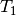amplitude.

ADC — How to cache quantities within the DPD library

• Type: integer
• Default: 2
CACHELEVEL (CCDENSITY)

CCDENSITY — The amount of cacheing of data to perform

• Type: integer
• Default: 2
CACHELEVEL (CCENERGY)

CCENERGY — Cacheing level for libdpd governing the storage of amplitudes, integrals, and intermediates in the CC procedure. A value of 0 retains no quantities in cache, while a level of 6 attempts to store all quantities in cache. For particularly large calculations, a value of 0 may help with certain types of memory problems. The default is 2, which means that all four-index quantites with up to two virtual-orbital indices (e.g.,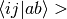integrals) may be held in the cache.

• Type: integer
• Default: 2
CACHELEVEL (CCEOM)
• Type: integer
• Default: 2
CACHELEVEL (CCHBAR)
• Type: integer
• Default: 2
CACHELEVEL (CCLAMBDA)
• Type: integer
• Default: 2
CACHELEVEL (CCRESPONSE)

CCRESPONSE — Cacheing level for libdpd

• Type: integer
• Default: 2
CACHELEVEL (CCSORT)
• Type: integer
• Default: 2
CACHELEVEL (DCFT)

DCFT — Controls how to cache quantities within the DPD library

• Type: integer
• Default: 2
CACHELEVEL (MP2)

MP2 — The amount of cacheing of data to perform

• Type: integer
• Default: 2
CACHELEVEL (OMP2)

OMP2

• Type: integer
• Default: 2
CACHELEVEL (STABILITY)
• Type: integer
• Default: 2
CACHELEVEL (TRANSQT2)
• Type: integer
• Default: 2
CACHETYPE (CCENERGY)

CCENERGY — Selects the priority type for maintaining the automatic memory cache used by the libdpd codes. A value of LOW selects a “low priority” scheme in which the deletion of items from the cache is based on pre-programmed priorities. A value of LRU selects a “least recently used” scheme in which the oldest item in the cache will be the first one deleted.

• Type: string
• Possible Values: LOW, LRU
• Default: LOW
CACHETYPE (CCEOM)
• Type: string
• Possible Values: LOW, LRU
• Default: LRU
CACHETYPE (MP2)

MP2 — The criterion used to retain/release cached data

• Type: string
• Possible Values: LRU, LOW
• Default: LRU
CANONICALIZE_ACTIVE_FAVG (MCSCF)

MCSCF — Do canonicalize the active orbitals such that the average Fock matrix is diagonal?

CANONICALIZE_INACTIVE_FAVG (MCSCF)

MCSCF — Do canonicalize the inactive (DOCC and Virtual) orbitals such that the average Fock matrix is diagonal?

OPTKING — Do read Cartesian Hessian? Only for experts - use FULL_HESS_EVERY instead.

CAS_FILES_WRITE (CLAG)

CLAG — Do write the OEI, TEI, OPDM, TPDM, and Lagrangian files in canonical form, Pitzer order?

CC (DETCI)

DETCI — Do coupled-cluster computation?

CC3_FOLLOW_ROOT (CCEOM)

CCEOM — Do ?

CC_A_RAS3_MAX (DETCI)

DETCI — maximum number of alpha electrons in RAS III, for CC

• Type: integer
• Default: -1
CC_B_RAS3_MAX (DETCI)

DETCI — maximum number of beta electrons in RAS III, for CC

• Type: integer
• Default: -1
CC_EX_LEVEL (DETCI)

DETCI — The CC excitation level

• Type: integer
• Default: 2
CC_FIX_EXTERNAL (DETCI)

DETCI (Expert) — Do fix amplitudes involving RAS I or RAS IV? Useful in mixed MP2-CC methods.

CC_FIX_EXTERNAL_MIN (DETCI)

DETCI (Expert) — Number of external indices before amplitude gets fixed by CC_FIX_EXTERNAL. Experimental.

• Type: integer
• Default: 1
CC_MACRO (DETCI)

DETCI (Expert) — CC_MACRO = [ [ex_lvl, max_holes_I, max_parts_IV, max_I+IV], [ex_lvl, max_holes_I, max_parts_IV, max_I+IV], ... ] Optional additional restrictions on allowed exictations in coupled-cluster computations, based on macroconfiguration selection. For each sub-array, [ex_lvl, max_holes_I, max_parts_IV, max_I+IV], eliminate cluster amplitudes in which: [the excitation level (holes in I + II) is equal to ex_lvl] AND [there are more than max_holes_I holes in RAS I, there are more than max_parts_IV particles in RAS IV, OR there are more than max_I+IV quasiparticles in RAS I + RAS IV].

• Type: array
• Default: No Default
CC_MAXITER (OMP2)

OMP2

• Type: integer
• Default: 50
CC_MIXED (DETCI)

DETCI (Expert) — Do ignore block if num holes in RAS I and II is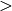cc_ex_lvl and if any indices correspond to RAS I or IV (i.e., include only all-active higher excitations)?

• Type: integer
• Default: 1

• Type: integer
• Default: 1

• Type: integer
• Default: 1

• Type: integer
• Default: 1
CC_OS_SCALE (CCENERGY)
• Type: double
• Default: 1.27
CC_RAS34_MAX (DETCI)

DETCI — maximum number of electrons in RAS III + IV, for CC

• Type: integer
• Default: -1
CC_RAS3_MAX (DETCI)

DETCI — maximum number of electrons in RAS III, for CC

• Type: integer
• Default: -1
CC_RAS4_MAX (DETCI)

DETCI — maximum number of electrons in RAS IV, for CC

• Type: integer
• Default: -1
CC_SS_SCALE (CCENERGY)
• Type: double
• Default: 1.13
CC_UPDATE_EPS (DETCI)

DETCI (Expert) — Do update T amplitudes with orbital eigenvalues? (Usually would do this). Not doing this is experimental.

CC_VAL_EX_LEVEL (DETCI)

DETCI — The CC valence excitation level

• Type: integer
• Default: 0
CC_VARIATIONAL (DETCI)

DETCI (Expert) — Do use variational energy expression in CC computation? Experimental.

DETCI — Do import a CC vector from disk?

CC_VECS_WRITE (DETCI)

DETCI — Do export a CC vector to disk?

CHECK_C_ORTHONORM (TRANSQT)

TRANSQT — Do ?

CI_DIIS (MCSCF)

MCSCF — Do use DIIS extrapolation to accelerate convergence of the CI coefficients?

DETCI — Number of threads for DETCI.

• Type: integer
• Default: 1
CIBLKS_PRINT (DETCI)

DETCI — Do print a summary of the CI blocks?

CPHF — Which states to save AD Matrices for? * Positive - Singlets * Negative - Triplets *

• Type: array
• Default: No Default
CIS_AMPLITUDE_CUTOFF (CPHF)

CPHF — Minimum singles amplitude to print in CIS analysis

• Type: double
• Default: 0.15
CIS_DOPDM_STATES (CPHF)

CPHF — Which states to save AO difference OPDMs for? * Positive - Singlets * Negative - Triplets *

• Type: array
• Default: No Default
CIS_MEM_SAFETY_FACTOR (CPHF)

CPHF — Memory safety factor for allocating JK

• Type: double
• Default: 0.75
CIS_NO_STATES (CPHF)

CPHF — Which states to save AO Natural Orbitals for? * Positive - Singlets * Negative - Triplets *

• Type: array
• Default: No Default
CIS_OPDM_STATES (CPHF)

CPHF — Which states to save AO OPDMs for? * Positive - Singlets * Negative - Triplets *

• Type: array
• Default: No Default
CIS_TOPDM_STATES (CPHF)

CPHF — Which states to save AO transition OPDMs for? * Positive - Singlets * Negative - Triplets *

• Type: array
• Default: No Default
COLLAPSE_SIZE (DETCI)

DETCI — Gives the number of vectors to retain when the Davidson subspace is collapsed (see MAX_NUM_VECS). If greater than one, the collapsed subspace retains the best estimate of the CI vector for the previous n iterations. Defaults to 1.

• Type: integer
• Default: 1
COLLAPSE_WITH_LAST (CCEOM)

CCEOM — Do ?

COMPLEX_TOLERANCE (CCEOM)
CONSECUTIVE_BACKSTEPS (OPTKING)

OPTKING — Set number of consecutive backward steps allowed in optimization

• Type: integer
• Default: 0
CORR_ANSATZ (PSIMRCC)

PSIMRCC — The ansatz to use for MRCC computations

• Type: string
• Possible Values: SR, MK, BW, APBW
• Default: MK
CORR_CCSD_T (PSIMRCC)

PSIMRCC — The type of CCSD(T) computation to perform

• Type: string
• Possible Values: STANDARD, PITTNER
• Default: STANDARD
CORR_CHARGE (PSIMRCC)

PSIMRCC — The molecular charge of the target state

• Type: integer
• Default: 0
CORR_MULTP (PSIMRCC)

PSIMRCC — The multiplicity,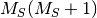, of the target state. Must be specified if different from the reference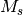.

• Type: integer
• Default: 1
CORR_REFERENCE (PSIMRCC)

PSIMRCC — Reference wavefunction type used in MRCC computations

• Type: string
• Possible Values: RHF, ROHF, TCSCF, MCSCF, GENERAL
• Default: GENERAL
CORR_WFN (PSIMRCC)

PSIMRCC — The type of correlated wavefunction

• Type: string
• Possible Values: PT2, CCSD, MP2-CCSD, CCSD_T
• Default: CCSD
COUPLING (PSIMRCC)

PSIMRCC — The order of coupling terms to include in MRCCSDT computations

• Type: string
• Possible Values: NONE, LINEAR, QUADRATIC, CUBIC
• Default: CUBIC
COUPLING_TERMS (PSIMRCC)

PSIMRCC — Do include the terms that couple the reference determinants?

COVALENT_CONNECT (OPTKING)

OPTKING — When determining connectivity, a bond is assigned if interatomic distance is less than (this number) * sum of covalent radii.

• Type: double
• Default: 1.3
CPHF_MEM_SAFETY_FACTOR (CPHF)

CPHF — Memory safety factor for allocating JK

• Type: double
• Default: 0.75

CPHF — Which tasks to run CPHF For * Valid choices: * -Polarizability *

• Type: array
• Default: No Default
CUTOFF (OMP2)

OMP2

• Type: integer
• Default: 14
D_CONVERGENCE (MCSCF)

MCSCF — Convergence criterion for density.

D_CONVERGENCE (SAPT)

SAPT — Convergence criterion for residual of the CPHF coefficients in the SAPT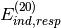term.

D_CONVERGENCE (SCF)

SCF — Convergence criterion for SCF density.

DAMPING_CONVERGENCE (SCF)

SCF — The density convergence threshold after which damping is no longer performed, if it is enabled. It is recommended to leave damping on until convergence, which is the default.

DAMPING_PERCENTAGE (DCFT)

DCFT — The amount (percentage) of damping to apply to the orbital update procedure: 0 will result in a full update, 100 will completely stall the update. A value around 20 (which corresponds to 20% of the previous iteration’s density being mixed into the current iteration) can help in cases where oscillatory convergence is observed.

• Type: double
• Default: 0.0
DAMPING_PERCENTAGE (PSIMRCC)

PSIMRCC — The amount (percentage) of damping to apply to the amplitude updates. 0 will result in a full update, 100 will completely stall the update. A value around 20 (which corresponds to 20% of the amplitudes from the previous iteration being mixed into the current iteration) can help in cases where oscillatory convergence is observed.

• Type: double
• Default: 0.0
DAMPING_PERCENTAGE (SCF)

SCF — The amount (percentage) of damping to apply to the early density updates. 0 will result in a full update, 100 will completely stall the update. A value around 20 (which corresponds to 20% of the previous iteration’s density being mixed into the current density) could help to solve problems with oscillatory convergence.

• Type: double
• Default: 100.0
DEBUG (CPHF)

CPHF — The amount of debug information printed to the output file

• Type: integer
• Default: 0
DEBUG (GLOBALS)

GLOBALS (Expert) — The amount of information to print to the output file

• Type: integer
• Default: 0
DELETE_AO (TRANSQT)

TRANSQT — Don’t ?

DELETE_RESTR_DOCC (TRANSQT)

TRANSQT — Don’t ?

DELETE_TEI (TRANSQT2)

TRANSQT2 — Boolean to delete the SO-basis two-electron integral file after the transformation

DELETE_TPDM (TRANSQT)

TRANSQT — Don’t ?

DENOMINATOR_ALGORITHM (SAPT)

SAPT — Denominator algorithm for PT methods. Laplace transformations are slightly more efficient.

• Type: string
• Possible Values: LAPLACE, CHOLESKY
• Default: LAPLACE
DENOMINATOR_DELTA (SAPT)

SAPT — Maximum error allowed (Max error norm in Delta tensor) in the approximate energy denominators employed for most of the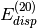and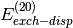evaluation.

• Type: double
• Default: 1.0e-6
DERTYPE (GLOBALS)

GLOBALS (Expert) — Derivative level

• Type: string
• Possible Values: NONE, FIRST, SECOND, RESPONSE
• Default: NONE
DETCI_FREEZE_CORE (DETCI)

DETCI — Do freeze core orbitals?

DF_BASIS_ELST (SAPT)

SAPT — Auxiliary basis set for SAPT Elst10 and Exch10 density fitting computations, may be important if heavier elements are involved. Defaults to BASIS-RI.

• Type: string
• Possible Values: basis string
• Default: No Default
DF_BASIS_MP2 (DFMP2)

DFMP2 — Auxiliary basis set for MP2 density fitting computations. Defaults to BASIS-RI.

• Type: string
• Possible Values: basis string
• Default: No Default
DF_BASIS_MP2 (LMP2)

LMP2 — Auxiliary basis set for MP2 density fitting calculations

• Type: string
• Possible Values: basis string
• Default: No Default
DF_BASIS_SAPT (SAPT)

SAPT — Auxiliary basis set for SAPT density fitting computations. Defaults to BASIS-RI.

• Type: string
• Possible Values: basis string
• Default: No Default
DF_BASIS_SCF (CPHF)

CPHF — Auxiliary basis for SCF

• Type: string
• Possible Values: basis string
• Default: No Default
DF_BASIS_SCF (SCF)

SCF — Auxiliary basis set for SCF density fitting computations. Defaults to BASIS-JKFIT.

• Type: string
• Possible Values: basis string
• Default: No Default
DF_FITTING_CONDITION (SCF)

SCF (Expert) — Fitting Condition

• Type: double
• Default: 1.0e-12
DF_INTS_IO (DFMP2)

DFMP2 (Expert) — IO caching for CP corrections, etc

• Type: string
• Possible Values: NONE, SAVE, LOAD
• Default: NONE
DF_INTS_IO (SCF)

SCF (Expert) — IO caching for CP corrections, etc

• Type: string
• Possible Values: NONE, SAVE, LOAD
• Default: NONE

DFMP2 — Number of threads to compute integrals with. 0 is wild card

• Type: integer
• Default: 0

SCF — Number of threads for integrals (may be turned down if memory is an issue). 0 is blank

• Type: integer
• Default: 0
DF_LMP2 (LMP2)

LMP2 — Do use density fitting? Turned on with specification of fitting basis.

DFMP2_MEM_FACTOR (DFMP2)

DFMP2 — % of memory for DF-MP2 three-index buffers

• Type: double
• Default: 0.9
DFT_ALPHA (SCF)

SCF — The DFT Exact-exchange parameter

• Type: double
• Default: 0.0
DFT_BASIS_TOLERANCE (SCF)

SCF — DFT basis cutoff.

DFT_BLOCK_MAX_POINTS (SCF)

SCF (Expert) — The maximum number of grid points per evaluation block.

• Type: integer
• Default: 5000

SCF (Expert) — The maximum radius to terminate subdivision of an octree block [au].

• Type: double
• Default: 3.0
DFT_BLOCK_MIN_POINTS (SCF)

SCF (Expert) — The minimum number of grid points per evaluation block.

• Type: integer
• Default: 1000
DFT_BLOCK_SCHEME (SCF)

SCF (Expert) — The blocking scheme for DFT.

• Type: string
• Possible Values: NAIVE, OCTREE
• Default: OCTREE

• Type: double
• Default: 1.0
DFT_CUSTOM_FUNCTIONAL (SCF)

SCF — A custom DFT functional object (built by Python or NULL/None)

• Type: python
• Default: No Default
DFT_FUNCTIONAL (SCF)

SCF — The DFT combined functional name, e.g. B3LYP, or GEN to use a python reference to a custom functional specified by DFT_CUSTOM_FUNCTIONAL.

• Type: string
• Default: No Default
DFT_GRID_NAME (SCF)

SCF (Expert) — The DFT grid specification, such as SG1.

• Type: string
• Possible Values: SG1
• Default: No Default
DFT_NUCLEAR_SCHEME (SCF)

SCF — Nuclear Scheme.

• Type: string
• Possible Values: TREUTLER, BECKE, NAIVE, STRATMANN
• Default: TREUTLER
DFT_OMEGA (SCF)

SCF — The DFT Range-separation parameter

• Type: double
• Default: 0.0
DFT_PRUNING_ALPHA (SCF)

SCF (Expert) — Spread alpha for logarithmic pruning.

• Type: double
• Default: 1.0
DFT_PRUNING_SCHEME (SCF)

SCF (Expert) — Pruning Scheme.

• Type: string
• Possible Values: FLAT, P_GAUSSIAN, D_GAUSSIAN, P_SLATER, D_SLATER, LOG_GAUSSIAN, LOG_SLATER
• Default: FLAT

SCF — Number of radial points.

• Type: integer
• Default: 99

• Type: string
• Possible Values: TREUTLER, BECKE, MULTIEXP, EM, MURA
• Default: TREUTLER
DFT_SPHERICAL_POINTS (SCF)

SCF — Number of spherical points (A Lebedev number).

• Type: integer
• Default: 302
DFT_SPHERICAL_SCHEME (SCF)

SCF — Spherical Scheme.

• Type: string
• Possible Values: LEBEDEV
• Default: LEBEDEV
DIAG_METHOD (CIS)

CIS

• Type: string
• Possible Values: DAVIDSON, FULL
• Default: DAVIDSON
DIAG_METHOD (DETCI)

DETCI — This specifies which method is to be used in diagonalizing the Hamiltonian. The valid options are: RSP, to form the entire H matrix and diagonalize using libciomr to obtain all eigenvalues (n.b. requires HUGE memory); OLSEN, to use Olsen’s preconditioned inverse subspace method (1990); MITRUSHENKOV, to use a 2x2 Olsen/Davidson method; and DAVIDSON (or SEM) to use Liu’s Simultaneous Expansion Method, which is identical to the Davidson method if only one root is to be found. There also exists a SEM debugging mode, SEMTEST. The SEM method is the most robust, but it also requires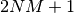CI vectors on disk, where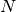is the maximum number of iterations and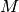is the number of roots.

• Type: string
• Possible Values: RSP, OLSEN, MITRUSHENKOV, DAVIDSON, SEM, SEMTEST
• Default: SEM
DIAGONAL_CCSD_T (PSIMRCC)

PSIMRCC — Do include the diagonal corrections in (T) computations?

DIAGONALIZE_HEFF (PSIMRCC)

PSIMRCC — Do diagonalize the effective Hamiltonian?

DIIS (CCENERGY)

CCENERGY — Do use DIIS extrapolation to accelerate convergence?

DIIS (CCLAMBDA)

CCLAMBDA — Do use DIIS extrapolation to accelerate convergence?

DIIS (CCRESPONSE)

CCRESPONSE — Do use DIIS extrapolation to accelerate convergence?

DIIS (DETCI)

DETCI — Do use DIIS extrapolation to accelerate CC convergence?

DIIS (LMP2)

LMP2 — Do use DIIS extrapolation to accelerate convergence?

DIIS (MCSCF)

MCSCF — Do use DIIS extrapolation to accelerate convergence of the SCF energy (MO coefficients only)?

DIIS (SCF)

SCF — Do use DIIS extrapolation to accelerate convergence?

DIIS_FREQ (DETCI)

DETCI — How often to do a DIIS extrapolation. 1 means do DIIS every iteration, 2 is every other iteration, etc.

• Type: integer
• Default: 1
DIIS_MAX_VECS (DCFT)

DCFT — Maximum number of error vectors stored for DIIS extrapolation

• Type: integer
• Default: 6
DIIS_MAX_VECS (DETCI)

DETCI — Maximum number of error vectors stored for DIIS extrapolation

• Type: integer
• Default: 5
DIIS_MAX_VECS (LMP2)

LMP2 — Maximum number of error vectors stored for DIIS extrapolation

• Type: integer
• Default: 5
DIIS_MAX_VECS (MCSCF)

MCSCF — Maximum number of error vectors stored for DIIS extrapolation

• Type: integer
• Default: 7
DIIS_MAX_VECS (OMP2)

OMP2 — Number of vectors used in DIIS

• Type: integer
• Default: 4
DIIS_MAX_VECS (PSIMRCC)

PSIMRCC — Maximum number of error vectors stored for DIIS extrapolation

• Type: integer
• Default: 7
DIIS_MAX_VECS (SCF)

SCF — Maximum number of error vectors stored for DIIS extrapolation

• Type: integer
• Default: 10
DIIS_MIN_VECS (DCFT)

DCFT — Minimum number of error vectors stored for DIIS extrapolation

• Type: integer
• Default: 3
DIIS_MIN_VECS (DETCI)

DETCI — Minimum number of error vectors stored for DIIS extrapolation

• Type: integer
• Default: 2
DIIS_MIN_VECS (SCF)

SCF — Minimum number of error vectors stored for DIIS extrapolation

• Type: integer
• Default: 2
DIIS_START (PSIMRCC)

PSIMRCC — The number of DIIS vectors needed before extrapolation is performed

• Type: integer
• Default: 2
DIIS_START (SCF)

SCF — The minimum iteration to start storing DIIS vectors

• Type: integer
• Default: 1
DIIS_START_CONVERGENCE (DCFT)

DCFT — Value of RMS of the density cumulant residual and SCF error vector below which DIIS extrapolation starts. Same keyword controls the DIIS extrapolation for the solution of the response equations.

DIIS_START_ITER (DETCI)

DETCI — Iteration at which to start using DIIS

• Type: integer
• Default: 1
DIIS_START_ITER (LMP2)

LMP2 — Iteration at which to start DIIS extrapolation

• Type: integer
• Default: 3
DIPMOM (DETCI)

DETCI — Do compute the dipole moment?

DISP_SIZE (FINDIF)

FINDIF — Displacement size in au for finite-differences.

• Type: double
• Default: 0.005
DISTANT_PAIR_CUTOFF (LMP2)

LMP2 — Distant pair cutoff

• Type: double
• Default: 8.0
DISTRIBUTED_MATRIX (SCF)

SCF (Expert) — The dimension sizes of the distributed matrix

• Type: array
• Default: No Default
DO_ALL_TEI (TRANSQT)

TRANSQT — Do ?

DO_SCS (OMP2)

OMP2 — Do ?

DO_SINGLETS (CPHF)

CPHF — Do singlet states? Default true

DO_SOS (OMP2)

OMP2 — Do ?

DO_THIRD_ORDER (SAPT)

SAPT (Expert) — Do compute third-order corrections?

DO_TRIPLETS (CPHF)

CPHF — Do triplet states? Default true

DOCC (GLOBALS)

GLOBALS — An array containing the number of doubly-occupied orbitals per irrep (in Cotton order)

• Type: array
• Default: No Default
DOCC (MCSCF)

MCSCF — The number of doubly occupied orbitals, per irrep

• Type: array
• Default: No Default
DOMAIN_PRINT (CIS)

CIS — Do ?

DOMAIN_PRINT_EXIT (LMP2)

LMP2 — Do exit after printing the domains?

DOMAINS (CIS)

CIS

• Type: array
• Default: No Default
E_CONVERGENCE (CCEOM)

CCEOM — Convergence criterion for excitation energy (change) in the Davidson algorithm for CC-EOM.

E_CONVERGENCE (DETCI)

DETCI — Convergence criterion for energy.

E_CONVERGENCE (LMP2)

LMP2 — Convergence criterion for energy (change).

E_CONVERGENCE (MCSCF)

MCSCF — Convergence criterion for energy.

E_CONVERGENCE (MRCC)

MRCC — This becomes tol (option #16) in fort.56.

E_CONVERGENCE (OMP2)

OMP2

E_CONVERGENCE (PSIMRCC)

PSIMRCC — Convergence criterion for energy.

E_CONVERGENCE (SAPT)

SAPT — Convergence criterion for energy (change) in the SAPTterm during solution of the CPHF equations.

E_CONVERGENCE (SCF)

SCF — Convergence criterion for SCF energy.

EOM_GUESS (CCEOM)

CCEOM — Specifies a set of single-excitation guess vectors for the EOM-CC procedure. If EOM_GUESS = SINGLES, the guess will be taken from the singles-singles block of the similarity-transformed Hamiltonian, Hbar. If EOM_GUESS = DISK, guess vectors from a previous computation will be read from disk. If EOM_GUESS = INPUT, guess vectors will be specified in user input. The latter method is not currently available.

• Type: string
• Possible Values: SINGLES, DISK, INPUT
• Default: SINGLES
EOM_REFERENCE (CCEOM)
• Type: string
• Possible Values: RHF, ROHF, UHF
• Default: RHF
EOM_REFERENCE (CCHBAR)
• Type: string
• Default: RHF
EOM_REFERENCE (CCSORT)
• Type: string
• Default: RHF
EX_ALLOW (DETCI)

DETCI (Expert) — An array of length EX_LEVEL specifying whether each excitation type (S,D,T, etc.) is allowed (1 is allowed, 0 is disallowed). Used to specify non-standard CI spaces such as CIST.

• Type: array
• Default: No Default
EX_LEVEL (DETCI)

DETCI — The CI excitation level

• Type: integer
• Default: 2
EXCITATION_RANGE (CCEOM)

CCEOM (Expert) — The depth into the occupied and valence spaces from which one-electron excitations are seeded into the Davidson guess to the CIS (the default of 2 includes all single excitations between HOMO-1, HOMO, LUMO, and LUMO+1). This CIS is in turn the Davidson guess to the EOM-CC. Expand to capture more exotic excited states in the EOM-CC calculation

• Type: integer
• Default: 2
EXPLICIT_HAMILTONIAN (CPHF)

CPHF — Do explicit hamiltonian only?

EXTERN (SCF)

SCF — An ExternalPotential (built by Python or NULL/None)

• Type: python
• Default: No Default
FAVG (MCSCF)

MCSCF — Do use the average Fock matrix during the SCF optimization?

FAVG_CCSD_T (PSIMRCC)

PSIMRCC — Do use the averaged Fock matrix over all references in (T) computations?

FAVG_START (MCSCF)

MCSCF — Iteration at which to begin using the averaged Fock matrix

• Type: integer
• Default: 5
FCI (DETCI)

DETCI — Do a full CI (FCI)? If TRUE, overrides the value of EX_LEVEL.

FCI_STRINGS (DETCI)

DETCI (Expert) — Do store strings specifically for FCI? (Defaults to TRUE for FCI.)

FILTER_GUESS (DETCI)

DETCI (Expert) — Do invoke the FILTER_GUESS options that are used to filter out some trial vectors which may not have the appropriate phase convention between two determinants? This is useful to remove, e.g., delta states when a sigma state is desired. The user inputs two determinants (by giving the absolute alpha string number and beta string number for each), and also the desired phase between these two determinants for guesses which are to be kept. FILTER_GUESS = TRUE turns on the filtering routine. Requires additional keywords FILTER_GUESS_DET1, FILTER_GUESS_DET2, and FILTER_GUESS_SIGN.

FILTER_GUESS_DET1 (DETCI)

DETCI (Expert) — Array specifying the absolute alpha string number and beta string number for the first determinant in the filter procedure. (See FILTER_GUESS).

• Type: array
• Default: No Default
FILTER_GUESS_DET2 (DETCI)

DETCI (Expert) — Array specifying the absolute alpha string number and beta string number for the second determinant in the filter procedure. (See FILTER_GUESS).

• Type: array
• Default: No Default
FILTER_GUESS_SIGN (DETCI)

DETCI (Expert) — The required phase (1 or -1) between the two determinants specified by FILTER_GUESS_DET1 and FILTER_GUESS_DET2.

• Type: integer
• Default: 1
FILTER_ZERO_DET (DETCI)

DETCI (Expert) — If present, the code will try to filter out a particular determinant by setting its CI coefficient to zero. FILTER_ZERO_DET = [alphastr, betastr] specifies the absolute alpha and beta string numbers of the target determinant. This could be useful for trying to exclude states that have a nonzero CI coefficient for the given determinant. However, this option was experimental and may not be effective.

• Type: array
• Default: No Default
FINAL_GEOM_WRITE (OPTKING)

OPTKING — Do save and print the geometry from the last projected step at the end of a geometry optimization? Otherwise (and by default), save and print the previous geometry at which was computed the gradient that satisfied the convergence criteria.

FIRST_TMP_FILE (TRANSQT)
• Type: integer
• Default: 150
FITTING_ALGORITHM (CPHF)

CPHF — Fitting algorithm (0 for old, 1 for new)

• Type: integer
• Default: 0
FITTING_CONDITION (CPHF)

CPHF — The maximum reciprocal condition allowed in the fitting metric

• Type: double
• Default: 1.0e-12
FLEXIBLE_G_CONVERGENCE (OPTKING)

OPTKING — Even if a user-defined threshold is set, allow for normal, flexible convergence criteria

FOCK_TOLERANCE (LMP2)

LMP2 — Minimum absolute value below which parts of the Fock matrix are skipped.

STABILITY — Do follow the most negative eigenvalue of the Hessian towards a lower energy HF solution? Follow a UHFUHF instability of same symmetry?

FOLLOW_ROOT (CLAG)

CLAG — Root to get OPDM

• Type: integer
• Default: 1
FOLLOW_ROOT (DETCI)

DETCI — The root to write out the two-particle density matrix for (the one-particle density matrices are written for all roots). Useful for a state-specific CASSCF or CI optimization on an excited state.

• Type: integer
• Default: 1
FOLLOW_ROOT (MCSCF)

MCSCF — Which solution of the SCF equations to find, where 1 is the SCF ground state

• Type: integer
• Default: 1
FOLLOW_ROOT (PSIMRCC)

PSIMRCC — Which root of the effective hamiltonian is the target state?

• Type: integer
• Default: 1
FOLLOW_VECTOR (DETCI)

DETCI (Expert) — In following a particular root (see FOLLOW_ROOT), sometimes the root number changes. To follow a root of a particular character, one can specify a list of determinants and their coefficients, and the code will follow the root with the closest overlap. The user specifies arrays containing the absolute alpha string indices (A_i below), absolute beta indices (B_i below), and CI coefficients (C_i below) to form the desired vector. The format is FOLLOW_VECTOR = [ [[A_1, B_1], C_1], [[A_2, B_2], C_2], ...].

• Type: array
• Default: No Default
FORCE_RESTART (CCENERGY)

CCENERGY (Expert) — Do restart the coupled-cluster iterations even if MO phases are screwed up?

FORCE_TWOCON (MCSCF)

MCSCF — Do attempt to force a two configruation solution by starting with CI coefficents of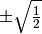?

FRAC_DIIS (SCF)

SCF — Do use DIIS extrapolation to accelerate convergence in frac?

SCF — Do recompute guess from stored orbitals?

FRAC_OCC (SCF)

SCF — The absolute indices of occupied orbitals to fractionally occupy (+/- for alpha/beta)

• Type: array
• Default: No Default
FRAC_RENORMALIZE (SCF)

SCF — Do renormalize C matrices prior to writing to checkpoint?

FRAC_START (SCF)

SCF — The iteration to start fractionally occupying orbitals (or 0 for no fractional occupation)

• Type: integer
• Default: 0
FRAC_VAL (SCF)

SCF — The occupations of the orbital indices specified above (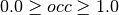)

• Type: array
• Default: No Default
FRAG_MODE (OPTKING)

OPTKING — For multi-fragment molecules, treat as single bonded molecule or via interfragment coordinates. A primary difference is that in MULTI mode, the interfragment coordinates are not redundant.

• Type: string
• Possible Values: SINGLE, MULTI
• Default: SINGLE
FREEZE_CORE (GLOBALS)

GLOBALS — Specifies how many core orbitals to freeze in correlated computations. TRUE will default to freezing the standard default number of core orbitals. For heavier elements, there can be some ambiguity in how many core orbitals to freeze; in such cases, SMALL picks the most conservative standard setting (freezes fewer orbitals), and LARGE picks the least conservative standard setting (freezes more orbitals). More precise control over the number of frozen orbitals can be attained by using the keywords NUM_FROZEN_DOCC (gives the total number of orbitals to freeze, program picks the lowest-energy orbitals) or FROZEN_DOCC (gives the number of orbitals to freeze per irreducible representation)

• Type: string
• Possible Values: FALSE, TRUE, SMALL, LARGE
• Default: FALSE
FREEZE_CORE (SAPT)

SAPT — The scope of core orbitals to freeze in evaluation of SAPTandterms. Recommended true for all SAPT computations

• Type: string
• Possible Values: FALSE, TRUE, SMALL, LARGE
• Default: FALSE
FREEZE_INTERFRAG (OPTKING)

OPTKING — Do freeze all interfragment modes?

FREEZE_INTRAFRAG (OPTKING)

OPTKING — Do freeze all fragments rigid?

FROZEN_DOCC (GLOBALS)

GLOBALS — An array containing the number of frozen doubly-occupied orbitals per irrep (these are not excited in a correlated wavefunction, nor can they be optimized in MCSCF

• Type: array
• Default: No Default
FROZEN_DOCC (PSIMRCC)

PSIMRCC — The number of frozen occupied orbitals per irrep

• Type: array
• Default: No Default
FROZEN_UOCC (GLOBALS)

GLOBALS — An array containing the number of frozen unoccupied orbitals per irrep (these are not populated in a correlated wavefunction, nor can they be optimized in MCSCF

• Type: array
• Default: No Default
FROZEN_UOCC (PSIMRCC)

PSIMRCC — The number of frozen virtual orbitals per irrep

• Type: array
• Default: No Default
FULL_HESS_EVERY (OPTKING)

OPTKING — Frequency with which to compute the full Hessian in the course of a geometry optimization. 0 means to compute the initial Hessian only, 1 means recompute every step, and N means recompute every N steps. The default (-1) is to never compute the full Hessian.

• Type: integer
• Default: -1
FULL_MATRIX (CCEOM)

CCEOM — Do ?

FZC_A_FILE (TRANSQT)
• Type: integer
• Default: PSIF_OEI
FZC_B_FILE (TRANSQT)
• Type: integer
• Default: PSIF_OEI
FZC_FILE (TRANSQT)
• Type: integer
• Default: PSIF_OEI
G_CONVERGENCE (OPTKING)

OPTKING — Set of optimization criteria. Specification of any MAX_*_G_CONVERGENCE or RMS_*_G_CONVERGENCE options will append to overwrite the criteria set here unless FLEXIBLE_G_CONVERGENCE is also on. See Table Geometry Convergence for details.

• Type: string
• Possible Values: QCHEM, MOLPRO, GAU, GAU_LOOSE, GAU_TIGHT, GAU_VERYTIGHT, TURBOMOLE, CFOUR, NWCHEM_LOOSE
• Default: QCHEM
GAUGE (CCDENSITY)

CCDENSITY — The type of gauge to use for properties

• Type: string
• Default: LENGTH
GAUGE (CCRESPONSE)

CCRESPONSE — Specifies the choice of representation of the electric dipole operator. Acceptable values are LENGTH for the usual length-gauge representation, VELOCITY for the modified velocity-gauge representation in which the static-limit optical rotation tensor is subtracted from the frequency- dependent tensor, or BOTH. Note that, for optical rotation calculations, only the choices of VELOCITY or BOTH will yield origin-independent results.

• Type: string
• Possible Values: LENGTH, VELOCITY, BOTH
• Default: LENGTH
GEOM_MAXITER (OPTKING)

OPTKING — Maximum number of geometry optimization steps

• Type: integer
• Default: 20
GUESS (SCF)

SCF — The type of guess orbitals

• Type: string
• Default: CORE
GUESS_VECTOR (DETCI)

DETCI (Expert) — Guess vector type. Accepted values are UNIT for a unit vector guess (NUM_ROOTS and NUM_INIT_VECS must both be 1); H0_BLOCK to use eigenvectors from the H0 BLOCK submatrix (default); DFILE to use NUM_ROOTS previously converged vectors in the D file; IMPORT to import a guess previously exported from a CI computation (possibly using a different CI space)

• Type: string
• Possible Values: UNIT, H0_BLOCK, DFILE, IMPORT
• Default: H0_BLOCK
H0_BLOCK_COUPLING (DETCI)

DETCI (Expert) — Do use coupling block in preconditioner?

H0_BLOCK_COUPLING_SIZE (DETCI)

DETCI (Expert) — Parameters which specifies the size of the coupling block within the generalized davidson preconditioner.

• Type: integer
• Default: 0
H0_BLOCKSIZE (DETCI)

DETCI (Expert) — This parameter specifies the size of the H0 block of the Hamiltonian which is solved exactly. The n determinants with the lowest SCF energy are selected, and a submatrix of the Hamiltonian is formed using these determinants. This submatrix is used to accelerate convergence of the CI iterations in the OLSEN and MITRUSHENKOV iteration schemes, and also to find a good starting guess for the SEM method if GUESS_VECTOR is H0_BLOCK. Defaults to 400. Note that the program may change the given size for Ms=0 cases (MS0 is TRUE) if it determines that the H0 block includes only one member of a pair of determinants related by time reversal symmetry. For very small block sizes, this could conceivably eliminate the entire H0 block; the program should print warnings if this occurs.

• Type: integer
• Default: 400
H0_GUESS_SIZE (DETCI)

DETCI (Expert) — size of H0 block for initial guess

• Type: integer
• Default: 400
H_BOND_CONNECT (OPTKING)

OPTKING — For now, this is a general maximum distance for the definition of H-bonds

• Type: double
• Default: 4.3
HD_AVG (DETCI)

DETCI (Expert) — How to average H diag energies over spin coupling sets. HD_EXACT uses the exact diagonal energies which results in expansion vectors which break spin symmetry. HD_KAVE averages the diagonal energies over a spin-coupling set yielding spin pure expansion vectors. ORB_ENER employs the sum of orbital energy approximation giving spin pure expansion vectors but usually doubles the number of Davidson iterations. EVANGELISTI uses the sums and differences of orbital energies with the SCF reference energy to produce spin pure expansion vectors. LEININGER approximation which subtracts the one-electron contribution from the orbital energies, multiplies by 0.5, and adds the one-electron contribution back in, producing spin pure expansion vectors and developed by Matt Leininger and works as well as EVANGELISTI.

• Type: string
• Possible Values: EVANGELISTI, HD_EXACT, HD_KAVE, ORB_ENER, LEININGER, Z_KAVE
• Default: EVANGELISTI
HD_OTF (DETCI)

DETCI (Expert) — Do compute the diagonal elements of the Hamiltonian matrix on-the-fly? Otherwise, a diagonal element vector is written to a separate file on disk.

HEFF4 (PSIMRCC)

PSIMRCC — Do include the fourth-order contributions to the effective Hamiltonian?

HEFF_PRINT (PSIMRCC)

PSIMRCC — Do print the effective Hamiltonian?

HESS_TYPE (OMP2)

OMP2

• Type: string
• Possible Values: NONE
• Default: NONE
HESS_UPDATE (OPTKING)

OPTKING — Hessian update scheme

• Type: string
• Possible Values: NONE, BFGS, MS, POWELL, BOFILL
• Default: BFGS
HESS_UPDATE_LIMIT (OPTKING)

OPTKING — Do limit the magnitude of changes caused by the Hessian update?

HESS_UPDATE_LIMIT_MAX (OPTKING)

OPTKING — If HESS_UPDATE_LIMIT is true, changes to the Hessian from the update are limited to the larger of HESS_UPDATE_LIMIT_SCALE * (the previous value) and HESS_UPDATE_LIMIT_MAX [au].

• Type: double
• Default: 1.00
HESS_UPDATE_LIMIT_SCALE (OPTKING)

OPTKING — If HESS_UPDATE_LIMIT is true, changes to the Hessian from the update are limited to the larger of HESS_UPDATE_LIMIT_SCALE * (the previous value) and HESS_UPDATE_LIMIT_MAX [au].

• Type: double
• Default: 0.50
HESS_UPDATE_USE_LAST (OPTKING)

OPTKING — Number of previous steps to use in Hessian update, 0 uses all

• Type: integer
• Default: 1
ICORE (DETCI)

DETCI — Specifies how to handle buffering of CI vectors. A value of 0 makes the program perform I/O one RAS subblock at a time; 1 uses entire CI vectors at a time; and 2 uses one irrep block at a time. Values of 0 or 2 cause some inefficiency in the I/O (requiring multiple reads of the C vector when constructing H in the iterative subspace if DIAG_METHOD = SEM), but require less core memory.

• Type: integer
• Default: 1
IGNORE_TAU (DCFT)

DCFT — Controls whether to ignore terms containing non-idempotent contribution to OPDM or not (for debug puproses only). For practical applications only the default must be used

INTCO_FIXED_EQ_FORCE_CONSTANT (OPTKING)

OPTKING — In constrained optimizations, for internal coordinates with user-specified equilibrium values, this is the force constant (in au) used to apply an additional force to each coordinate. If the user is only concerned to satify the desired constraint, then the user need only ensure that this value is sufficiently large. Alternatively, the user may specify this value to apply a force of a particular magnitude, in which case the given equilibrium value may or may not be reached by the optimization.

• Type: double
• Default: 2.0
INTCOS_GENERATE_EXIT (OPTKING)

OPTKING — Do only generate the internal coordinates and then stop?

INTERFRAG_DIST_INV (OPTKING)

OPTKING — Do use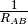for the stretching coordinate between fragments? Otherwise, use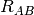.

INTERFRAG_HESS (OPTKING)

OPTKING — Model Hessian to guess interfragment force constants

• Type: string
• Possible Values: DEFAULT, FISCHER_LIKE
• Default: DEFAULT
INTERFRAG_MODE (OPTKING)

OPTKING — When interfragment coordinates are present, use as reference points either principal axes or fixed linear combinations of atoms.

• Type: string
• Possible Values: FIXED, INTERFRAGMENT
• Default: FIXED
INTERFRAG_STEP_LIMIT (OPTKING)

OPTKING — Maximum step size in bohr or radian along an interfragment coordinate

• Type: double
• Default: 0.4
INTERNAL_ROTATIONS (MCSCF)

MCSCF — Do ?

INTRAFRAG_HESS (OPTKING)

OPTKING — Model Hessian to guess intrafragment force constants

• Type: string
• Possible Values: FISCHER, SCHLEGEL, SIMPLE
• Default: SCHLEGEL
INTRAFRAG_STEP_LIMIT (OPTKING)

OPTKING — Initial maximum step size in bohr or radian along an internal coordinate

• Type: double
• Default: 0.4
INTRAFRAG_STEP_LIMIT_MAX (OPTKING)

OPTKING — Upper bound for dynamic trust radius [au]

• Type: double
• Default: 1.0
INTRAFRAG_STEP_LIMIT_MIN (OPTKING)

OPTKING — Lower bound for dynamic trust radius [au]

• Type: double
• Default: 0.001
INTS_TOLERANCE (CCDENSITY)

CCDENSITY — Minimum absolute value below which integrals are neglected.

INTS_TOLERANCE (CCSORT)

CCSORT — Minimum absolute value below which integrals are neglected.

INTS_TOLERANCE (DCFT)

DCFT — Minimum absolute value below which integrals are neglected

INTS_TOLERANCE (DFMP2)

DFMP2 — Minimum absolute value below which integrals are neglected.

INTS_TOLERANCE (LMP2)

LMP2 — Minimum absolute value below which integrals are neglected.

INTS_TOLERANCE (MRCC)

MRCC — Minimum absolute value below which integrals are neglected.

INTS_TOLERANCE (SAPT)

SAPT — Minimum absolute value below which all three-index DF integrals and those contributing to four-index integrals are neglected. The default is conservative, but there isn’t much to be gained from loosening it, especially for higher-order SAPT.

INTS_TOLERANCE (SCF)

SCF — Minimum absolute value below which TEI are neglected.

INTS_TOLERANCE (TRANSQT)

TRANSQT — Minimum absolute value below which integrals are neglected.

INTS_TOLERANCE (TRANSQT2)

TRANSQT2 — Minimum absolute value below which integrals are neglected.

IRC_DIRECTION (OPTKING)

OPTKING — IRC mapping direction

• Type: string
• Possible Values: FORWARD, BACKWARD
• Default: FORWARD
IRC_STEP_SIZE (OPTKING)

OPTKING — IRC step size in bohr(amu)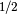.

• Type: double
• Default: 0.2
IRC_STOP (OPTKING)

OPTKING — Decide when to stop IRC calculations

• Type: string
• Possible Values: ASK, STOP, GO
• Default: STOP
ISTOP (DETCI)

DETCI — Do stop DETCI after string information is formed and before integrals are read?

IVO (TRANSQT)

TRANSQT — Do ?

J_FILE (TRANSQT)
• Type: integer
• Default: 91
JOBTYPE (CCLAMBDA)

CCLAMBDA (Expert) — Type of job being performed

• Type: string
• Default: No Default
JOBTYPE (MP2)

MP2 (Expert) — Type of job being performed

• Type: string
• Default: SP
KEEP_INTCOS (OPTKING)

OPTKING — Keep internal coordinate definition file.

KEEP_J (TRANSQT)

TRANSQT — Do keep half-transformed integrals?

KEEP_OEIFILE (CCSORT)

CCSORT — Do retain the input one-electron integrals?

KEEP_PRESORT (TRANSQT)

TRANSQT — Do ?

KEEP_TEIFILE (CCSORT)

CCSORT — Do retain the input two-electron integrals?

LAG_IN_FILE (TRANSQT)
• Type: integer
• Default: PSIF_MO_LAG
LAGRAN_DOUBLE (TRANSQT)

TRANSQT — Do ?

LAGRAN_HALVE (TRANSQT)

TRANSQT — Do ?

LAMBDA_MAXITER (DCFT)

DCFT — Maximum number of density cumulant update micro-iterations per macro-iteration (for ALOGRITHM = TWOSTEP). Same keyword controls the maximum number of density cumulant response micro-iterations per macro-iteration for the solution of the response equations (for RESPONSE_ALOGRITHM = TWOSTEP)

• Type: integer
• Default: 50
LEVEL_SHIFT (MCSCF)

MCSCF — Level shift to aid convergence

• Type: double
• Default: 0.0
LEVEL_SHIFT (OMP2)

OMP2

• Type: double
• Default: 0.02
LINEAR (CCRESPONSE)

CCRESPONSE — Do Bartlett size-extensive linear model?

LOCAL (CCENERGY)

CCENERGY — Do simulate the effects of local correlation techniques?

LOCAL (CCEOM)

CCEOM — Do ?

LOCAL (CCLAMBDA)

CCLAMBDA — Do ?

LOCAL (CCRESPONSE)

CCRESPONSE — Do simulate local correlation?

LOCAL (CCSORT)

CCSORT — Do ?

LOCAL (CIS)

CIS — Do ?

LOCAL_AMPS_PRINT_CUTOFF (CIS)

CIS

• Type: double
• Default: 0.60
LOCAL_CORE_CUTOFF (CCSORT)
• Type: double
• Default: 0.05
LOCAL_CPHF_CUTOFF (CCLAMBDA)
• Type: double
• Default: 0.10
LOCAL_CPHF_CUTOFF (CCRESPONSE)
• Type: double
• Default: 0.10
LOCAL_CPHF_CUTOFF (CCSORT)
• Type: double
• Default: 0.10
LOCAL_CUTOFF (CCENERGY)

CCENERGY — Value (always between one and zero) for the Broughton-Pulay completeness check used to contruct orbital domains for local-CC calculations. See J. Broughton and P. Pulay, J. Comp. Chem. 14, 736-740 (1993) and C. Hampel and H.-J. Werner, J. Chem. Phys. 104, 6286-6297 (1996).

• Type: double
• Default: 0.02
LOCAL_CUTOFF (CCEOM)
• Type: double
• Default: 0.02
LOCAL_CUTOFF (CCLAMBDA)
• Type: double
• Default: 0.02
LOCAL_CUTOFF (CCRESPONSE)
• Type: double
• Default: 0.01
LOCAL_CUTOFF (CCSORT)
• Type: double
• Default: 0.02
LOCAL_CUTOFF (CIS)

CIS

• Type: double
• Default: 0.02
LOCAL_CUTOFF (LMP2)

LMP2 — Localization cutoff

• Type: double
• Default: 0.02
LOCAL_DO_SINGLES (CCEOM)

CCEOM — Do ?

LOCAL_DOMAIN_MAG (CCSORT)

CCSORT — Do ?

LOCAL_DOMAIN_POLAR (CCSORT)

CCSORT — Do ?

LOCAL_DOMAIN_SEP (CCSORT)

CCSORT — Do ?

LOCAL_FILTER_SINGLES (CCEOM)

CCEOM — Do ?

LOCAL_FILTER_SINGLES (CCLAMBDA)

CCLAMBDA — Do ?

LOCAL_FILTER_SINGLES (CCRESPONSE)

CCRESPONSE — Do ?

LOCAL_FILTER_SINGLES (CCSORT)

CCSORT — Do ?

LOCAL_GHOST (CCEOM)
• Type: integer
• Default: -1
LOCAL_GHOST (CIS)

CIS

• Type: integer
• Default: -1
LOCAL_METHOD (CCENERGY)

CCENERGY — Type of local-CCSD scheme to be simulated. WERNER selects the method developed by H.-J. Werner and co-workers, and AOBASIS selects the method developed by G.E. Scuseria and co-workers (currently inoperative).

• Type: string
• Possible Values: WERNER, AOBASIS
• Default: WERNER
LOCAL_METHOD (CCEOM)
• Type: string
• Possible Values: WERNER, AOBASIS
• Default: WERNER
LOCAL_METHOD (CCLAMBDA)
• Type: string
• Default: WERNER
LOCAL_METHOD (CCRESPONSE)
• Type: string
• Default: WERNER
LOCAL_METHOD (CCSORT)
• Type: string
• Default: WERNER
LOCAL_METHOD (CIS)

CIS

• Type: string
• Possible Values: AOBASIS, WERNER
• Default: WERNER
LOCAL_PAIRDEF (CCENERGY)
• Type: string
• Possible Values: BP, RESPONSE
• Default: BP
LOCAL_PAIRDEF (CCLAMBDA)
• Type: string
• Default: No Default
LOCAL_PAIRDEF (CCRESPONSE)
• Type: string
• Default: NONE
LOCAL_PAIRDEF (CCSORT)
• Type: string
• Default: BP
LOCAL_PRECONDITIONER (CCEOM)
• Type: string
• Possible Values: HBAR, FOCK
• Default: HBAR
LOCAL_WEAKP (CCENERGY)

CCENERGY — Desired treatment of “weak pairs” in the local-CCSD method. A value of NEGLECT ignores weak pairs entirely. A value of NONE treats weak pairs in the same manner as strong pairs. A value of MP2 uses second-order perturbation theory to correct the local-CCSD energy computed with weak pairs ignored.

• Type: string
• Possible Values: NONE, NEGLECT, MP2
• Default: NONE
LOCAL_WEAKP (CCEOM)
• Type: string
• Possible Values: NONE, MP2, NEGLECT
• Default: NONE
LOCAL_WEAKP (CCLAMBDA)
• Type: string
• Default: NONE
LOCAL_WEAKP (CCRESPONSE)
• Type: string
• Default: NONE
LOCAL_WEAKP (CCSORT)
• Type: string
• Default: NONE
LOCAL_WEAKP (CIS)

CIS

• Type: string
• Possible Values: MP2, NEGLECT, NONE
• Default: MP2
LOCK_OCC (DCFT)

DCFT — Controls whether to force the occupation to be that of the SCF guess. For practical applications only the default must be used

LOCK_SINGLET (PSIMRCC)

PSIMRCC — Do lock onto a singlet root?

LSE (DETCI)

DETCI — Do use least-squares extrapolation in iterative solution of CI vector?

LSE_COLLAPSE (DETCI)

DETCI — Number of iterations between least-squares extrapolations

• Type: integer
• Default: 3
LSE_TOLERANCE (DETCI)

DETCI — Minimum converged energy for least-squares extrapolation to be performed

M_FILE (TRANSQT)
• Type: integer
• Default: 0

DFMP2 (Expert) — A helpful option, used only in debugging the MADNESS version

• Type: integer
• Default: 0
MAT_NUM_COLUMN_PRINT (GLOBALS)

GLOBALS (Expert) — Number of columns to print in calls to Matrix::print_mat.

• Type: integer
• Default: 5
MAX_BUCKETS (TRANSQT)
• Type: integer
• Default: 499
MAX_DISP_G_CONVERGENCE (OPTKING)

OPTKING — Convergence criterion for geometry optmization: maximum displacement (internal coordinates, atomic units).

MAX_ENERGY_G_CONVERGENCE (OPTKING)

OPTKING — Convergence criterion for geometry optmization: maximum energy change.

MAX_FORCE_G_CONVERGENCE (OPTKING)

OPTKING — Convergence criterion for geometry optmization: maximum force (internal coordinates, atomic units).

OMP2

MAX_NUM_VECS (DETCI)

DETCI — Maximum number of Davidson subspace vectors which can be held on disk for the CI coefficient and sigma vectors. (There is one H(diag) vector and the number of D vectors is equal to the number of roots). When the number of vectors on disk reaches the value of MAX_NUM_VECS, the Davidson subspace will be collapsed to COLLAPSE_SIZE vectors for each root. This is very helpful for saving disk space. Defaults to MAXITER * NUM_ROOTS + NUM_INIT_VECS.

• Type: integer
• Default: 0
MAXITER (CCENERGY)

CCENERGY — Maximum number of iterations to solve the CC equations

• Type: integer
• Default: 50
MAXITER (CCEOM)

CCEOM — Maximum number of iterations

• Type: integer
• Default: 80
MAXITER (CCLAMBDA)

CCLAMBDA — Maximum number of iterations

• Type: integer
• Default: 50
MAXITER (CCRESPONSE)

CCRESPONSE — Maximum number of iterations to converge perturbed amplitude equations

• Type: integer
• Default: 50
MAXITER (CIS)

CIS — Maximum number of iterations

• Type: integer
• Default: 500
MAXITER (DCFT)

DCFT — Maximum number of macro-iterations for both energy and the solution of the response equations

• Type: integer
• Default: 40
MAXITER (DETCI)

DETCI — Maximum number of iterations to diagonalize the Hamiltonian

• Type: integer
• Default: 12
MAXITER (LMP2)

LMP2 — Maximum number of iterations

• Type: integer
• Default: 50
MAXITER (MCSCF)

MCSCF — Maximum number of iterations

• Type: integer
• Default: 100
MAXITER (PSIMRCC)

PSIMRCC — Maximum number of iterations to determine the amplitudes

• Type: integer
• Default: 100
MAXITER (SAPT)

SAPT — Maxmum number of CPHF iterations

• Type: integer
• Default: 50
MAXITER (SCF)

SCF — Maximum number of iterations

• Type: integer
• Default: 100

ADC — The amount of memory available (in Mb)

• Type: integer
• Default: 1000
MEMORY (LMP2)

LMP2

• Type: integer
• Default: 2000
MIXED (DETCI)

DETCI (Expert) — Do allow “mixed” RAS II/RAS III excitations into the CI space? If FALSE, then if there are any electrons in RAS III, then the number of holes in RAS I cannot exceed the given excitation level EX_LEVEL.

MIXED4 (DETCI)

DETCI (Expert) — Do allow “mixed” excitations involving RAS IV into the CI space. Useful to specify a split-virtual CISD[TQ] computation. If FALSE, then if there are any electrons in RAS IV, then the number of holes in RAS I cannot exceed the given excitation level EX_LEVEL.

MO_MAXITER (OMP2)

OMP2

• Type: integer
• Default: 50

MCSCF — Do read in from file the MOs from a previous computation?

OMP2 — Do read coefficient matrices from psi files?

MO_RELAX (DCFT)

DCFT — Controls whether to relax the orbitals during the energy computation or not (for debug puproses only). For practical applications only the default must be used

MO_STEP_MAX (OMP2)

OMP2

• Type: double
• Default: 0.5
MO_WRITE (OMP2)

OMP2 — Do write coefficient matrices to psi files?

MODE (TRANSQT)
• Type: string
• Possible Values: TO_MO, TO_AO
• Default: TO_MO
MODULE (CPHF)

CPHF — What app to test?

• Type: string
• Possible Values: RCIS, RCPHF, RTDHF, RCPKS, RTDA, RTDDFT
• Default: RCIS
MOM_OCC (SCF)

SCF — The absolute indices of orbitals to excite from in MOM (+/- for alpha/beta)

• Type: array
• Default: No Default
MOM_START (SCF)

SCF — The iteration to start MOM on (or 0 for no MOM)

• Type: integer
• Default: 0
MOM_VIR (SCF)

SCF — The absolute indices of orbitals to excite to in MOM (+/- for alpha/beta)

• Type: array
• Default: No Default
MOORDER (TRANSQT)
• Type: array
• Default: No Default
MP2_AMPS_PRINT (CCENERGY)

CCENERGY — Do print the MP2 amplitudes which are the starting guesses for RHF and UHF reference functions?

MP2_CCSD_METHOD (PSIMRCC)

PSIMRCC — How to perform MP2_CCSD computations

• Type: string
• Possible Values: I, IA, II
• Default: II
MP2_GUESS (PSIMRCC)

PSIMRCC — Do start from a MP2 guess?

MP2_OS_SCALE (CCENERGY)
• Type: double
• Default: 1.20
MP2_OS_SCALE (DFMP2)

DFMP2 — OS Scale

• Type: double
• Default: 6.0/5.0
MP2_OS_SCALE (LMP2)

LMP2

• Type: double
• Default: 6.0/5.0
MP2_OS_SCALE (MP2)

MP2 — The scale factor used for opposite-spin pairs in SCS computations

• Type: double
• Default: 6.0/5.0
MP2_OS_SCALE (OMP2)

OMP2

• Type: double
• Default: 6.0/5.0
MP2_SS_SCALE (CCENERGY)
• Type: double
• Default: 1.0/3.0
MP2_SS_SCALE (DFMP2)

DFMP2 — SS Scale

• Type: double
• Default: 1.0/3.0
MP2_SS_SCALE (LMP2)

LMP2

• Type: double
• Default: 1.0/3.0
MP2_SS_SCALE (MP2)

MP2 — The scale factor used for same-spin pairs in SCS computations

• Type: double
• Default: 1.0/3.0
MP2_SS_SCALE (OMP2)

OMP2

• Type: double
• Default: 1.0/3.0
MP2R12A (TRANSQT)
• Type: string
• Possible Values: MP2R12AERI, MP2R12AR12, MP2R12AR12T1
• Default: MP2R12AERI
MPN (DETCI)

DETCI — Do compute the MPn series out to kth order where k is determined by MAX_NUM_VECS ? For open-shell systems (REFERENCE is ROHF, WFN is ZAPTN), DETCI will compute the ZAPTn series. GUESS_VECTOR must be set to UNIT, HD_OTF must be set to TRUE, and HD_AVG must be set to orb_ener; these should happen by default for MPN = TRUE.

MPN_ORDER_SAVE (DETCI)

DETCI (Expert) — If 0, save the MPn energy; if 1, save the MP(2n-1) energy (if available from MPN_WIGNER = true); if 2, save the MP(2n-2) energy (if available from MPN_WIGNER = true).

• Type: integer
• Default: 0
MPN_SCHMIDT (DETCI)

DETCI (Expert) — Do employ an orthonormal vector space rather than storing the kth order wavefunction?

MPN_WIGNER (DETCI)

DETCI (Expert) — Do use Wigner formulas in the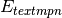series?

MRCC_LEVEL (MRCC)

MRCC — Maximum excitation level. This is used ONLY if it is explicity set by the user. Single-reference case: all excitations up to this level are included, e.g., 2 for CCSD, 3 for CCSDT, 4 for CCSDTQ, etc. This becomes ex.lev (option #1) in fort.56.

• Type: integer
• Default: 2
MRCC_METHOD (MRCC)

MRCC (Expert) — If more than one root is requested and calc=1, LR-CC (EOM-CC) calculation is performed automatically for the excited states. This overrides all automatic determination of method and will only work with energy(). This becomes CC/CI (option #5) in fort.56 | begin{tabular}{ccc} | Value & Method & Description \ | hline | 1 & CC & \ | 2 & CC(n-1)[n] & \ | 3 & CC(n-1)(n) & (CC(n-1)[n] energy is also calculated) \ | 4 & CC(n-1)(n)_L & (CC(n-1)[n] and CC(n-1)(n) energies are also calculated) \ | 5 & CC(n)-1a & \ | 6 & CC(n)-1b & \ | 7 & CCn & \ | 8 & CC(n)-3 & \ | end{tabular}

• Type: integer
• Default: 1
MRCC_NUM_SINGLET_ROOTS (MRCC)

MRCC — Number of singlet roots. (Strictly speaking number of of roots with M_s=0 and S is even.) Use this option only with closed shell reference determinant, it must be zero otherwise. This becomes nsing (option #2) in fort.56.

• Type: integer
• Default: 1
MRCC_NUM_TRIPLET_ROOTS (MRCC)

MRCC — Number of triplet roots. (Strictly speaking number of of roots with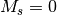and S is odd.) See notes at option MRCC_NUM_SINGLET_ROOTS. This becomes ntrip (option #3) in fort.56.

• Type: integer
• Default: 0

MRCC (Expert) — Sets the OMP_NUM_THREADS environment variable before calling MRCC. If the environment variable OMP_NUM_THREADS is set prior to calling PSI4 then that value is used. When set, this option overrides everything. Be aware the -n command-line option described in section Threading does not affect MRCC.

• Type: integer
• Default: 1
MRCC_RESTART (MRCC)

MRCC (Expert) — The program restarts from the previously calculated parameters if it is 1. In case it is 2, the program executes automatically the lower-level calculations of the same type consecutively (e.g., CCSD, CCSDT, and CCSDTQ if CCSDTQ is requested) and restarts each calculation from the previous one (rest=2 is available only for energy calculations). Currently, only a value of 0 and 2 are supported. This becomes rest (option #4) in fort.56.

• Type: integer
• Default: 0
MS0 (DETCI)

DETCI — Do use thecomponent of the state? Defaults to TRUE if closed-shell and FALSE otherwise. Related to the S option.

NAT_ORBS (OMP2)

OMP2 — Do ?

NAT_ORBS (SAPT)

SAPT — Do natural orbitals to speed up evaluation of the triples contribution to dispersion by truncating the virtual orbital space? Recommended true for all SAPT computations.

NAT_ORBS_T2 (SAPT)

SAPT — Do use MP2 natural orbital approximations for the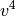block of two-electron integrals in the evaluation of second-order T2 amplitudes? This approximation is promising for accuracy and computational savings, but it has not been rigorously tested.

NAT_ORBS_WRITE (DETCI)

DETCI — Do write the natural orbitals?

NAT_ORBS_WRITE_ROOT (DETCI)

DETCI — Sets the root number for which CI natural orbitals are written to PSIF_CHKPT. The default value is 1 (lowest root).

• Type: integer
• Default: 1
NEGLECT_DISTANT_PAIR (LMP2)

LMP2 — Do neglect distant pairs?

NEW_TRIPLES (CCENERGY)

CCENERGY — Do ?

NEW_TRIPLES (CCEOM)

CCEOM — Do ?

ADC — The convergence criterion for pole searching step.

NO_DFILE (DETCI)

DETCI (Expert) — Do use the last vector space in the BVEC file to write scratch DVEC rather than using a separate DVEC file? (Only possible if NUM_ROOTS = 1.)

NO_RESPONSE (SAPT)

SAPT — Don’t solve the CPHF equations? Evaluate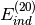and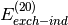instead of their response-including coupterparts. Only turn on this option if the induction energy is not going to be used.

NO_SINGLES (PSIMRCC)

PSIMRCC — Do ?

ADC — The cutoff norm of residual vector in SEM step.

ADC — Number of components of transition amplitudes printed

• Type: integer
• Default: 5
NUM_AMPS_PRINT (CCENERGY)

CCENERGY — Number of important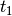and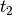amplitudes to print

• Type: integer
• Default: 10
NUM_AMPS_PRINT (CCEOM)

CCEOM — Number of important CC amplitudes to print

• Type: integer
• Default: 5
NUM_AMPS_PRINT (CCLAMBDA)
• Type: integer
• Default: 10
NUM_AMPS_PRINT (CCRESPONSE)
• Type: integer
• Default: 5
NUM_AMPS_PRINT (DETCI)

DETCI — Number of important CC amplitudes per excitation level to print. CC analog to NUM_DETS_PRINT.

• Type: integer
• Default: 10
NUM_DETS_PRINT (DETCI)

DETCI — Number of important determinants to print

• Type: integer
• Default: 20
NUM_FROZEN_DOCC (GLOBALS)

GLOBALS — The number of core orbitals to freeze in later correlated computations. FROZEN_DOCC trumps this option

• Type: integer
• Default: 0
NUM_FROZEN_UOCC (GLOBALS)

GLOBALS — The number of virtual orbitals to freeze in later correlated computations. FROZEN_UOCC trumps this option

• Type: integer
• Default: 0
NUM_INIT_VECS (DETCI)

DETCI (Expert) — The number of initial vectors to use in the CI iterative procedure. Defaults to the number of roots.

• Type: integer
• Default: 0
NUM_ROOTS (DETCI)

DETCI — number of CI roots to find

• Type: integer
• Default: 1
NUM_VECS_PRINT (STABILITY)

STABILITY — Number of lowest MO Hessian eigenvalues to print

• Type: integer
• Default: 0
NUM_VECS_WRITE (DETCI)

DETCI — Number of vectors to export

• Type: integer
• Default: 1
OCC_TOLERANCE (SAPT)

SAPT — Minimum occupation (eigenvalues of the MP2 OPDM) below which virtual natural orbitals are discarded for evaluating the triples contribution to dispersion.

OEI_A_FILE (TRANSQT)
• Type: integer
• Default: PSIF_OEI
OEI_B_FILE (TRANSQT)
• Type: integer
• Default: PSIF_OEI
OEI_FILE (TRANSQT)
• Type: integer
• Default: PSIF_OEI
OFFDIAGONAL_CCSD_T (PSIMRCC)

PSIMRCC — Do include the off-diagonal corrections in (T) computations?

OMEGA (CCRESPONSE)

CCRESPONSE — Array that specifies the desired frequencies of the incident radiation field in CCLR calculations. If only one element is given, the units will be assumed to be atomic units. If more than one element is given, then the units must be specified as the final element of the array. Acceptable units are HZ, NM, EV, and AU.

• Type: array
• Default: No Default
OMEGA (CCSORT)

CCSORT — Energy of applied field [au] for dynamic properties

• Type: array
• Default: No Default
OMEGA (RESPONSE)
• Type: array
• Default: No Default
OMP2_ORBS_PRINT (OMP2)

OMP2 — Do ?

• Type: integer
• Default: 0
ONEPDM (CCDENSITY)

CCDENSITY — Do ?

OPDM (DETCI)

DETCI — Do compute one-particle density matrix if not otherwise required?

OPDM (MP2)

MP2 — Do compute the one particle density matrix, for properties?

OPDM_AVG (DETCI)

DETCI — Do average the OPDM over several roots in order to obtain a state-average one-particle density matrix? This density matrix can be diagonalized to obtain the CI natural orbitals.

OPDM_IN_FILE (TRANSQT)
• Type: integer
• Default: PSIF_MO_OPDM
OPDM_KE (DETCI)

DETCI (Expert) — Do compute the kinetic energy contribution from the correlated part of the one-particle density matrix?

OPDM_OUT_FILE (TRANSQT)
• Type: integer
• Default: PSIF_AO_OPDM
OPDM_PRINT (DETCI)

DETCI — Do print the one-particle density matrix for each root?

OPDM_RELAX (CCDENSITY)

CCDENSITY — Do relax the one-particle density matrix?

OPDM_RELAX (MP2)

MP2 — Do add relaxation terms to the one particle density matrix, for properties?

OPT_METHOD (OMP2)

OMP2

• Type: string
• Possible Values: SD, DIIS
• Default: DIIS
OPT_TYPE (OPTKING)

OPTKING — Specifies minimum search, transition-state search, or IRC following

• Type: string
• Possible Values: MIN, TS, IRC
• Default: MIN
ORTH_TYPE (OMP2)

OMP2

• Type: string
• Possible Values: GS, MGS
• Default: MGS
PAIR_ENERGIES_PRINT (CCENERGY)

CCENERGY — Do print MP2 and CCSD pair energies for RHF references?

PARALLEL (SCF)

SCF (Expert) — Do run in parallel?

PERTURB_CBS (PSIMRCC)

PSIMRCC (Expert) — Do compute the perturbative corrections for basis set incompleteness?

PERTURB_CBS_COUPLING (PSIMRCC)

PSIMRCC (Expert) — Do include the terms that couple different reference determinants in perturbative CBS correction computations?

PERTURB_H (SCF)

SCF — Do perturb the Hamiltonian?

PERTURB_MAGNITUDE (DETCI)

DETCI (Expert) — The magnitude of perturbation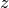in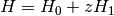• Type: double
• Default: 1.0
PERTURB_MAGNITUDE (SCF)

SCF — Size of the perturbation

• Type: double
• Default: 0.0
PERTURB_WITH (SCF)

SCF — The operator used to perturb the Hamiltonian, if requested

• Type: string
• Possible Values: DIPOLE_X, DIPOLE_Y, DIPOLE_Z
• Default: DIPOLE_X
PITZER (TRANSQT)

TRANSQT — Do ?

POINTS (FINDIF)

FINDIF — Number of points for finite-differences (3 or 5)

• Type: integer
• Default: 3

ADC — Maximum iteration number in pole searching

• Type: integer
• Default: 20

ADC — Do use the partial renormalization scheme for the ground state wavefunction?

PRECONDITIONER (DETCI)

DETCI — This specifies the type of preconditioner to use in the selected diagonalization method. The valid options are: DAVIDSON which approximates the Hamiltonian matrix by the diagonal elements; H0BLOCK_INV which uses an exact Hamiltonian of H0_BLOCKSIZE and explicitly inverts it; GEN_DAVIDSON which does a spectral decomposition of H0BLOCK; ITER_INV using an iterative approach to obtain the correction vector of H0BLOCK. The H0BLOCK_INV, GEN_DAVIDSON, and ITER_INV approaches are all formally equivalent but the ITER_INV is less computationally expensive. Default is DAVIDSON.

• Type: string
• Possible Values: LANCZOS, DAVIDSON, GEN_DAVIDSON, H0BLOCK, H0BLOCK_INV, ITER_INV, H0BLOCK_COUPLING, EVANGELISTI
• Default: DAVIDSON
PRESORT_FILE (TRANSQT)
• Type: integer
• Default: PSIF_SO_PRESORT
PRINT (CPHF)

CPHF — The amount of information printed to the output file

• Type: integer
• Default: 1
PRINT (GLOBALS)

GLOBALS — The amount of information to print to the output file. 1 prints basic information, and higher levels print more information. A value of 5 will print very large amounts of debugging information.

• Type: integer
• Default: 1
PRINT (SAPT)

SAPT — The amount of information to print to the output file for the sapt module. For 0, only the header and final results are printed. For 1, (recommended for large calculations) some intermediate quantities are also printed.

• Type: integer
• Default: 1
PRINT_LVL (TRANSQT)
• Type: integer
• Default: 1
PRINT_MOS (TRANSQT)

TRANSQT — Do ?

PRINT_OE_INTEGRALS (TRANSQT)

TRANSQT — Do ?

PRINT_REORDER (TRANSQT)

TRANSQT — Do ?

PRINT_SORTED_OE_INTS (TRANSQT)

TRANSQT — Do ?

PRINT_SORTED_TE_INTS (TRANSQT)

TRANSQT — Do ?

PRINT_TE_INTEGRALS (TRANSQT)

TRANSQT — Do ?

PRINT_TEI (TRANSQT2)

TRANSQT2 — Do ?

PROCESS_GRID (SCF)

SCF (Expert) — The dimension sizes of the processor grid

• Type: array
• Default: No Default
PROP_ALL (CCDENSITY)

CCDENSITY — Compute non-relaxed properties for all excited states.

PROP_ALL (CCLAMBDA)

CCLAMBDA — Compute unrelaxed properties for all excited states.

PROP_ROOT (CCDENSITY)
• Type: integer
• Default: 1
PROP_ROOT (CCEOM)

CCEOM — Root number (within its irrep) for computing properties. Defaults to highest root requested.

• Type: integer
• Default: 0
PROP_ROOT (CCLAMBDA)
• Type: integer
• Default: 1
PROP_SYM (CCDENSITY)

CCDENSITY — The symmetry of states

• Type: integer
• Default: 1
PROP_SYM (CCEOM)

CCEOM — Symmetry of the state to compute properties. Defaults to last irrep for which states are requested.

• Type: integer
• Default: 1
PROP_SYM (CCLAMBDA)
• Type: integer
• Default: 1
PROPERTIES (GLOBALS)

GLOBALS — List of properties to compute

• Type: array
• Default: No Default
PROPERTY (CCENERGY)
• Type: string
• Possible Values: POLARIZABILITY, ROTATION, MAGNETIZABILITY, ROA, ALL
• Default: POLARIZABILITY
PROPERTY (CCRESPONSE)

CCRESPONSE — The response property desired. Acceptable values are POLARIZABILITY (default) for dipole-polarizabilities, ROTATION for specific rotations, ROA for Raman Optical Activity, and ALL for all of the above.

• Type: string
• Possible Values: POLARIZABILITY, ROTATION, ROA, ALL
• Default: POLARIZABILITY
PROPERTY (CCSORT)
• Type: string
• Default: POLARIZABILITY
PROPERTY (RESPONSE)

RESPONSE — Array that specifies the desired frequencies of the incident radiation field in CCLR calculations. If only one element is given, the units will be assumed to be atomic units. If more than one element is given, then the units must be specified as the final element of the array. Acceptable units are HZ, NM, EV, and AU.

• Type: string
• Possible Values: POLARIZABILITY, ROTATION, ROA, ALL
• Default: POLARIZABILITY
PSIMRCC (TRANSQT)

TRANSQT — Do ?

PT_ENERGY (PSIMRCC)

PSIMRCC — The type of perturbation theory computation to perform

• Type: string
• Possible Values: SECOND_ORDER, SCS_SECOND_ORDER, PSEUDO_SECOND_ORDER, SCS_PSEUDO_SECOND_ORDER
• Default: SECOND_ORDER
PUREAM (GLOBALS)

GLOBALS — Do use pure angular momentum basis functions? If not explicitly set, the default comes from the basis set.

QRHF (TRANSQT)

TRANSQT — Do ?

R4S (DETCI)

DETCI (Expert) — Do restrict strings with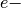in RAS IV? Useful to reduce the number of strings required if MIXED4=true, as in a split-virutal CISD[TQ] computation. If more than one electron is in RAS IV, then the holes in RAS I cannot exceed the number of particles in RAS III + RAS IV (i.e., EX_LEVEL), or else the string is discarded.

R_CONVERGENCE (CCENERGY)

CCENERGY — Convergence criterion for wavefunction (change) in CC amplitude equations.

R_CONVERGENCE (CCEOM)

CCEOM — Convergence criterion for norm of the residual vector in the Davidson algorithm for CC-EOM.

R_CONVERGENCE (CCLAMBDA)

CCLAMBDA — Convergence criterion for wavefunction (change) in CC lambda-amplitude equations.

R_CONVERGENCE (CCRESPONSE)

CCRESPONSE — Convergence criterion for wavefunction (change) in perturbed CC equations.

R_CONVERGENCE (CIS)

CIS — Convergence criterion for CIS wavefunction.

R_CONVERGENCE (DCFT)

DCFT — Convergence criterion for the RMS of the residual vector in the density cumulant updates as well as the solution of the density cumulant and orbital response equations. In the orbital updates controls the RMS of the SCF error vector

R_CONVERGENCE (DETCI)

DETCI — Convergence criterion for CI residual vector in the Davidson algorithm (RMS error). The default is 1e-4 for energies and 1e-7 for gradients.

R_CONVERGENCE (LMP2)

LMP2 — Convergence criterion for T2 amplitudes (RMS change).

R_CONVERGENCE (OMP2)

OMP2

R_CONVERGENCE (PSIMRCC)

PSIMRCC — Convergence criterion for amplitudes (residuals).

RAS1 (DETCI)

DETCI (Expert) — An array giving the number of orbitals per irrep for RAS1

• Type: array
• Default: No Default
RAS1 (TRANSQT)

TRANSQT (Expert) — An array giving the number of orbitals per irrep for RAS1

• Type: array
• Default: No Default
RAS2 (DETCI)

DETCI (Expert) — An array giving the number of orbitals per irrep for RAS2

• Type: array
• Default: No Default
RAS2 (TRANSQT)

TRANSQT (Expert) — An array giving the number of orbitals per irrep for RAS2

• Type: array
• Default: No Default
RAS3 (DETCI)

DETCI (Expert) — An array giving the number of orbitals per irrep for RAS3

• Type: array
• Default: No Default
RAS3 (TRANSQT)

TRANSQT (Expert) — An array giving the number of orbitals per irrep for RAS3

• Type: array
• Default: No Default
RAS34_MAX (DETCI)

DETCI — maximum number of electrons in RAS III + IV

• Type: integer
• Default: -1
RAS3_MAX (DETCI)

DETCI — maximum number of electrons in RAS III

• Type: integer
• Default: -1
RAS4 (DETCI)

DETCI (Expert) — An array giving the number of orbitals per irrep for RAS4

• Type: array
• Default: No Default
RAS4 (TRANSQT)

TRANSQT (Expert) — An array giving the number of orbitals per irrep for RAS4

• Type: array
• Default: No Default
RAS4_MAX (DETCI)

DETCI — maximum number of electrons in RAS IV

• Type: integer
• Default: -1

• Type: string
• Default: No Default
REFERENCE (CCDENSITY)

CCDENSITY — Reference wavefunction type

• Type: string
• Default: RHF
REFERENCE (CCENERGY)

CCENERGY — Reference wavefunction type

• Type: string
• Possible Values: RHF, ROHF, UHF
• Default: RHF
REFERENCE (CCEOM)

CCEOM — Reference wavefunction type

• Type: string
• Possible Values: RHF, ROHF, UHF
• Default: RHF
REFERENCE (CCRESPONSE)

CCRESPONSE — Reference wavefunction type

• Type: string
• Default: RHF
REFERENCE (CCSORT)

CCSORT — Reference wavefunction type

• Type: string
• Default: RHF
REFERENCE (CCTRIPLES)

CCTRIPLES — Reference wavefunction type

• Type: string
• Default: RHF
REFERENCE (CIS)

CIS — Reference wavefunction type

• Type: string
• Possible Values: RHF, ROHF, UHF
• Default: RHF
REFERENCE (DETCI)

DETCI — Reference wavefunction type

• Type: string
• Possible Values: RHF, ROHF
• Default: RHF
REFERENCE (LMP2)

LMP2 — Reference wavefunction type

• Type: string
• Possible Values: RHF
• Default: RHF
REFERENCE (MCSCF)

MCSCF — Reference wavefunction type

• Type: string
• Possible Values: RHF, ROHF, UHF, TWOCON, MCSCF, GENERAL
• Default: RHF
REFERENCE (MP2)

MP2 — Reference wavefunction type

• Type: string
• Possible Values: RHF, UHF, ROHF
• Default: RHF
REFERENCE (RESPONSE)

RESPONSE — Reference wavefunction type

• Type: string
• Default: RHF
REFERENCE (SCF)

SCF — Reference wavefunction type

• Type: string
• Possible Values: RHF, ROHF, UHF, CUHF, RKS, UKS
• Default: RHF
REFERENCE (STABILITY)

STABILITY — Reference wavefunction type

• Type: string
• Possible Values: RHF, UHF, ROHF
• Default: RHF
REFERENCE (TRANSQT)

TRANSQT — Reference wavefunction type

• Type: string
• Default: RHF
REFERENCE (TRANSQT2)

TRANSQT2 — Reference wavefunction type

• Type: string
• Default: RHF
REFERENCE_SYM (DETCI)

DETCI (Expert) — Irrep for CI vectors; -1 = find automatically. This option allows the user to look for CI vectors of a different irrep than the reference. This probably only makes sense for Full CI, and it would probably not work with unit vector guesses. Numbering starts from zero for the totally-symmetric irrep.

• Type: integer
• Default: -1
REORDER (TRANSQT)

TRANSQT — Do ?

REPL_OTF (DETCI)

DETCI (Expert) — Do string replacements on the fly in DETCI? Can save a gigantic amount of memory (especially for truncated CI’s) but is somewhat flaky and hasn’t been tested for a while. It may work only works for certain classes of RAS calculations. The current code is very slow with this option turned on.

RESPONSE_ALGORITHM (DCFT)

DCFT — The algorithm to use for the solution of the response equations for the analytic gradients and properties. Two-step algorithm is generally more efficient than simultaneous and is used by default

• Type: string
• Possible Values: TWOSTEP, SIMULTANEOUS
• Default: TWOSTEP
RESTART (CCENERGY)

CCENERGY — Do restart the coupled-cluster iterations from oldandamplitudes? For geometry optimizations, Brueckner calculations, etc. the iterative solution of the CC amplitude equations may benefit considerably by reusing old vectors as initial guesses. Assuming that the MO phases remain the same between updates, the CC codes will, by default, re-use old vectors, unless the user sets RESTART = false.

RESTART (CCLAMBDA)

CCLAMBDA — Do ?

RESTART (CCRESPONSE)

CCRESPONSE — Do restart from on-disk amplitudes?

RESTART (DETCI)

DETCI — Do restart a DETCI iteration that terminated prematurely? It assumes that the CI and sigma vectors are on disk; the number of vectors specified by RESTART_VECS (obsolete) is collapsed down to one vector per root.

RESTART_EOM_CC3 (CCEOM)

CCEOM — Do ?

RESTRICTED_DOCC (DETCI)

DETCI — An array giving the number of restricted doubly-occupied orbitals per irrep (not excited in CI wavefunctions, but orbitals can be optimized in MCSCF)

• Type: array
• Default: No Default
RESTRICTED_DOCC (PSIMRCC)

PSIMRCC — The number of doubly occupied orbitals per irrep

• Type: array
• Default: No Default
RESTRICTED_DOCC (TRANSQT)

TRANSQT — An array giving the number of restricted doubly-occupied orbitals per irrep (not excited in CI wavefunctions, but orbitals can be optimized in MCSCF)

• Type: array
• Default: No Default
RESTRICTED_UOCC (DETCI)

DETCI — An array giving the number of restricted unoccupied orbitals per irrep (not occupied in CI wavefunctions, but orbitals can be optimized in MCSCF)

• Type: array
• Default: No Default
RESTRICTED_UOCC (TRANSQT)

TRANSQT — An array giving the number of restricted unoccupied orbitals per irrep (not occupied in CI wavefunctions, but orbitals can be optimized in MCSCF)

• Type: array
• Default: No Default
RFO_FOLLOW_ROOT (OPTKING)

OPTKING — Do follow the initial RFO vector after the first step?

RFO_ROOT (OPTKING)

OPTKING — Root for RFO to follow, 0 being lowest (for a minimum)

• Type: integer
• Default: 0
RHF_TRIPLETS (CCEOM)

CCEOM — Do ?

RMS_DISP_G_CONVERGENCE (OPTKING)

OPTKING — Convergence criterion for geometry optmization: rms displacement (internal coordinates, atomic units).

RMS_FORCE_G_CONVERGENCE (OPTKING)

OPTKING — Convergence criterion for geometry optmization: rms force (internal coordinates, atomic units).

OMP2

ADC — The poles per irrep vector

• Type: array
• Default: No Default
ROOTS_PER_IRREP (CCDENSITY)

CCDENSITY — The number of electronic states to computed, per irreducible representation

• Type: array
• Default: No Default
ROOTS_PER_IRREP (CCEOM)

CCEOM — Number of excited states per irreducible representation for EOM-CC and CC-LR calculations. Irreps denote the final state symmetry, not the symmetry of the transition.

• Type: array
• Default: No Default
ROOTS_PER_IRREP (CCLAMBDA)
• Type: array
• Default: No Default
ROOTS_PER_IRREP (CIS)

CIS — The number of electronic states to computed, per irreducible representation

• Type: array
• Default: No Default
ROTATE_MO_ANGLE (MCSCF)

MCSCF (Expert) — For orbital rotations after convergence, the angle (in degrees) by which to rotate.

• Type: integer
• Default: 0
ROTATE_MO_IRREP (MCSCF)

MCSCF (Expert) — For orbital rotations after convergence, irrep (1-based, Cotton order) of the orbitals to rotate.

• Type: integer
• Default: 1
ROTATE_MO_P (MCSCF)

MCSCF (Expert) — For orbital rotations after convergence, number of the first orbital (1-based) to rotate.

• Type: integer
• Default: 1
ROTATE_MO_Q (MCSCF)

MCSCF (Expert) — For orbital rotations after convergence, number of the second orbital (1-based) to rotate.

• Type: integer
• Default: 2
ROTATION_SCHEME (STABILITY)

STABILITY — Method for following eigenvectors, either 0 by angles or 1 by antisymmetric matrix.

• Type: integer
• Default: 0
S (DETCI)

DETCI — The value of the spin quantum number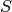is given by this option. The default is determined by the value of the multiplicity. This is used for two things: (1) determining the phase of the redundant half of the CI vector when thecomponent is used (i.e., MS0 = TRUE), and (2) making sure the guess vector has the desired value of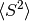(if S_SQUARED is TRUE and ICORE = 1).

• Type: double
• Default: 0.0
S_ORTHOGONALIZATION (SCF)

SCF — SO orthogonalization: symmetric or canonical?

• Type: string
• Possible Values: SYMMETRIC, CANONICAL
• Default: SYMMETRIC
S_SQUARED (DETCI)

DETCI — Do calculate the value offor each root?

S_TOLERANCE (SCF)

SCF — Minimum S matrix eigenvalue to be used before compensating for linear dependencies.

SCF (Expert) — SAD Guess Cholesky Cutoff (for eliminating redundancies).

SCF — Convergence criterion for SCF density in SAD Guess.

SCF — Convergence criterion for SCF energy in SAD Guess.

SCF (Expert) — SAD Guess F-mix Iteration Start

• Type: integer
• Default: 50

SCF (Expert) — Maximum number of SAD guess iterations

• Type: integer
• Default: 50

SCF (Expert) — The amount of SAD information to print to the output

• Type: integer
• Default: 0
SAPT (SCF)

SCF (Expert) — Are going to do SAPT? If so, what part?

• Type: string
• Possible Values: FALSE, 2-DIMER, 2-MONOMER_A, 2-MONOMER_B, 3-TRIMER, 3-DIMER_AB, 3-DIMER_BC, 3-DIMER_AC, 3-MONOMER_A, 3-MONOMER_B, 3-MONOMER_C
• Default: FALSE
SAPT_LEVEL (SAPT)

SAPT — The level of theory for SAPT

• Type: string
• Possible Values: SAPT0, SAPT2, SAPT2+, SAPT2+3
• Default: SAPT0
SAPT_MEM_CHECK (SAPT)

SAPT — Do force SAPT2 and higher to die if it thinks there isn’t enough memory? Turning this off is ill-advised.

SAPT_MEM_SAFETY (SAPT)

SAPT — Memory safety

• Type: double
• Default: 0.9
SAPT_OS_SCALE (SAPT)

SAPT — The scale factor used for opposite-spin pairs in SCS computations. SS/OS decomposition performed forandterms.

• Type: double
• Default: 6.0/5.0
SAPT_SS_SCALE (SAPT)

SAPT — The scale factor used for same-spin pairs in SCS computations. SS/OS decomposition performed forandterms.

• Type: double
• Default: 1.0/3.0
SAVE_JK (SCF)

SCF — Keep JK object for later use?

SCALE (STABILITY)

STABILITY — Scale factor (between 0 and 1) for orbital rotation step

• Type: double
• Default: 0.5
SCF_MAXITER (DCFT)

DCFT — Maximum number of orbital update micro-iterations per macro-iteration (for ALOGRITHM = TWOSTEP). Same keyword controls the maximum number of orbital response micro-iterations per macro-iteration for the solution of the response equations (for RESPONSE_ALOGRITHM = TWOSTEP)

• Type: integer
• Default: 50
SCF_MEM_SAFETY_FACTOR (SCF)

SCF — Memory safety factor for allocating JK

• Type: double
• Default: 0.75
SCF_TYPE (CPHF)

CPHF — SCF Type

• Type: string
• Possible Values: DIRECT, DF, PK, OUT_OF_CORE, PS
• Default: DIRECT
SCF_TYPE (SCF)

SCF — What algorithm to use for the SCF computation

• Type: string
• Possible Values: DIRECT, DF, PK, OUT_OF_CORE, PS
• Default: PK

CCEOM — Minimum absolute value above which a guess vector to a root is added to the Davidson algorithm in the EOM-CC iterative procedure.

SCHWARZ_CUTOFF (CPHF)

CPHF — The schwarz cutoff value

• Type: double
• Default: 1.0e-12
SCREEN_INTS (LMP2)

LMP2 — Do screen integrals?

SCS (LMP2)

LMP2 — Do ?

SCS (MP2)

MP2 — Do perform a spin component scaled MP2 computation?

SCS_CCSD (CCENERGY)

CCENERGY — Do ?

SCS_MP2 (CCENERGY)

CCENERGY — Do ?

SCS_N (LMP2)

LMP2 — Do ?

SCS_N (MP2)

MP2 — Do perform a spin component scaled (N) MP2 computation?

SCSN_MP2 (CCENERGY)

CCENERGY — Do ?

SEKINO (CCLAMBDA)

CCLAMBDA — Do ?

SEKINO (CCRESPONSE)

CCRESPONSE — Do Sekino-Bartlett size-extensive model-III?

ADC — Maximum iteration number in simultaneous expansion method

• Type: integer
• Default: 30
SEMICANONICAL (CCENERGY)

CCENERGY — Convert ROHF MOs to semicanonical MOs

SEMICANONICAL (CCEOM)

CCEOM — Convert ROHF MOs to semicanonical MOs

SEMICANONICAL (CCSORT)

CCSORT — Convert ROHF MOs to semicanonical MOs

SEMICANONICAL (CCTRIPLES)

CCTRIPLES — Convert ROHF MOs to semicanonical MOs

SEMICANONICAL (TRANSQT2)

TRANSQT2 — Convert ROHF MOs to semicanonical MOs

SF_RESTRICT (DETCI)

DETCI (Expert) — Do eliminate determinants not valid for spin-complete spin-flip CI’s? [see J. S. Sears et al, J. Chem. Phys. 118, 9084-9094 (2003)]

SIGMA_OVERLAP (DETCI)

DETCI (Expert) — Do print the sigma overlap matrix? Not generally useful.

SINGLES_PRINT (CCEOM)

CCEOM — Do print information on the iterative solution to the single-excitation EOM-CC problem used as a guess to full EOM-CC?

SMALL_CUTOFF (PSIMRCC)
• Type: integer
• Default: 0
SO_S_FILE (TRANSQT)
• Type: integer
• Default: PSIF_OEI
SO_T_FILE (TRANSQT)
• Type: integer
• Default: PSIF_OEI
SO_TEI_FILE (TRANSQT)
• Type: integer
• Default: PSIF_SO_TEI
SO_V_FILE (TRANSQT)
• Type: integer
• Default: PSIF_OEI
SOCC (GLOBALS)

GLOBALS — An array containing the number of singly-occupied orbitals per irrep (in Cotton order)

• Type: array
• Default: No Default
SOCC (MCSCF)

MCSCF — The number of singly occupied orbitals, per irrep

• Type: array
• Default: No Default
SOLVER_CONVERGENCE (CPHF)

CPHF — Solver convergence threshold (max 2-norm).

SOLVER_EXACT_DIAGONAL (CPHF)

CPHF — Solver exact diagonal or eigenvalue difference?

SOLVER_MAX_SUBSPACE (CPHF)

CPHF — DL Solver maximum number of subspace vectors

• Type: integer
• Default: 6
SOLVER_MAXITER (CPHF)

CPHF — Solver maximum iterations

• Type: integer
• Default: 100
SOLVER_MIN_SUBSPACE (CPHF)

CPHF — DL Solver number of subspace vectors to collapse to

• Type: integer
• Default: 2
SOLVER_N_GUESS (CPHF)

CPHF — DL Solver number of guesses

• Type: integer
• Default: 1
SOLVER_N_ROOT (CPHF)

CPHF — DL Solver number of roots

• Type: integer
• Default: 1
SOLVER_NORM (CPHF)

CPHF — DL Solver minimum corrector norm to add to subspace

• Type: double
• Default: 1.0e-6
SOLVER_PRECONDITION (CPHF)

CPHF — Solver precondition type

• Type: string
• Possible Values: SUBSPACE, JACOBI, NONE
• Default: JACOBI
SOLVER_PRECONDITION_MAXITER (CPHF)

CPHF — Solver precondtion max steps

• Type: integer
• Default: 1
SOLVER_PRECONDITION_STEPS (CPHF)

CPHF — Solver precondition step type

• Type: string
• Possible Values: CONSTANT, TRIANGULAR
• Default: TRIANGULAR
SOLVER_QUANTITY (CPHF)

CPHF — Solver residue or eigenvector delta

• Type: string
• Possible Values: EIGENVECTOR, RESIDUAL
• Default: RESIDUAL
SOLVER_TYPE (CPHF)

CPHF — Solver type (for interchangeable solvers)

• Type: string
• Possible Values: DL, RAYLEIGH
• Default: DL
SORTED_TEI_FILE (TRANSQT)
• Type: integer
• Default: PSIF_MO_TEI
SOS_SCALE (OMP2)

OMP2

• Type: double
• Default: 1.3
SOS_SCALE2 (OMP2)

OMP2

• Type: double
• Default: 1.2

CCENERGY — Do print spin-adapted pair energies?

SS_E_CONVERGENCE (CCEOM)

CCEOM — Convergence criterion for excitation energy (change) in the Davidson algorithm for the CIS guess to CC-EOM.

SS_R_CONVERGENCE (CCEOM)

CCEOM — Convergence criterion for norm of the residual vector in the Davidson algorithm for the CIS guess to CC-EOM.

SS_SKIP_DIAG (CCEOM)

CCEOM — Do ?

SS_VECS_PER_ROOT (CCEOM)
• Type: integer
• Default: 5
STEP_TYPE (OPTKING)

OPTKING — Geometry optimization step type, either Newton-Raphson or Rational Function Optimization

• Type: string
• Possible Values: RFO, NR, SD
• Default: RFO
T2_COUPLED (CCENERGY)

CCENERGY — Do ?

T3_WS_INCORE (CCENERGY)

CCENERGY — Do ?

T3_WS_INCORE (CCEOM)

CCEOM — Do ?

T_AMPS (CCHBAR)

CCHBAR — Do compute the Tamplitude equation matrix elements?

TAU_SQUARED (DCFT)

DCFT — Controls whether to compute the DCFT energy with the Tau^2 correction to Tau

TDHF_MEM_SAFETY_FACTOR (CPHF)

CPHF — Memory safety factor for allocating JK

• Type: double
• Default: 0.75
TDM (DETCI)

DETCI — Do compute the transition density? Note: only transition densities between roots of the same symmetry will be evaluated. DETCI does not compute states of different irreps within the same computation; to do this, lower the symmetry of the computation.

TDM_PRINT (DETCI)

DETCI — Do print the transition density?

TDM_WRITE (DETCI)

DETCI — Do write the transition density?

TEST_B (OPTKING)

OPTKING — Do test B matrix?

TEST_DERIVATIVE_B (OPTKING)

OPTKING — Do test derivative B matrix?

TIKHONOW_MAX (PSIMRCC)

PSIMRCC — The cycle after which Tikhonow regularization is stopped. Set to zero to allow regularization in all iterations

• Type: integer
• Default: 5
TIKHONOW_OMEGA (DCFT)

DCFT — The shift applied to the denominator in the density cumulant update iterations

• Type: double
• Default: 0.0
TIKHONOW_OMEGA (PSIMRCC)

PSIMRCC — The shift to apply to the denominators, {it c.f.} Taube and Bartlett, JCP, 130, 144112 (2009)

• Type: double
• Default: 0.0
TIKHONOW_TRIPLES (PSIMRCC)

PSIMRCC (Expert) — Do use Tikhonow regularization in (T) computations?

TILE_SZ (SCF)

SCF (Expert) — The tile size for the distributed matrices

• Type: integer
• Default: 512
TPDM (DCFT)

DCFT — Controls whether to compute unrelaxed two-particle density matrix at the end of the energy computation

TPDM (DETCI)

DETCI — Do compute two-particle density matrix if not otherwise required?

TRANSQT — Do ?

TPDM_FILE (TRANSQT)
• Type: integer
• Default: PSIF_MO_TPDM
TPDM_PRINT (DETCI)

DETCI — Do print the two-particle density matrix? (Warning: large tensor)

TRIPLES_ALGORITHM (PSIMRCC)

PSIMRCC — The type of algorithm to use for (T) computations

• Type: string
• Possible Values: SPIN_ADAPTED, RESTRICTED, UNRESTRICTED
• Default: RESTRICTED
TRIPLES_DIIS (PSIMRCC)

PSIMRCC — Do use DIIS extrapolation to accelerate convergence for iterative triples excitations?

TURN_ON_ACTV (MCSCF)
• Type: integer
• Default: 0
UNITS (GLOBALS)

GLOBALS — Units used in geometry specification

• Type: string
• Possible Values: BOHR, AU, A.U., ANGSTROMS, ANG, ANGSTROM
• Default: ANGSTROMS
UPDATE (DETCI)

DETCI — The update or correction vector formula, either DAVIDSON (default) or OLSEN.

• Type: string
• Possible Values: DAVIDSON, OLSEN
• Default: DAVIDSON
USE_SPIN_SYM (PSIMRCC)

PSIMRCC — Do use symmetry to map equivalent determinants onto each other, for efficiency?

USE_SPIN_SYMMETRY (PSIMRCC)

PSIMRCC (Expert) — Whether to use spin symmetry to map equivalent configurations onto each other, for efficiency

VAL_EX_LEVEL (DETCI)

DETCI — In a RAS CI, this is the additional excitation level for allowing electrons out of RAS I into RAS II. The maximum number of holes in RAS I is therefore EX_LEVEL + VAL_EX_LEVEL.

• Type: integer
• Default: 0
VECS_CC3 (CCEOM)
• Type: integer
• Default: 10
VECS_PER_ROOT (CCEOM)
• Type: integer
• Default: 12
VECS_WRITE (DETCI)

DETCI — Do store converged vector(s) at the end of the run? The vector(s) is(are) stored in a transparent format such that other programs can use it easily. The format is specified in psi4/src/lib/libqt/slaterdset.h .

WABEI_LOWDISK (CCHBAR)

CCHBAR — Do use the minimal-disk algorithm for Wabei? It’s VERY slow!

WFN (CCDENSITY)

CCDENSITY (Expert) — Wavefunction type

• Type: string
• Default: SCF
WFN (CCENERGY)

CCENERGY (Expert) — Wavefunction type

• Type: string
• Possible Values: CCSD, CCSD_T, EOM_CCSD, LEOM_CCSD, BCCD, BCCD_T, CC2, CC3, EOM_CC2, EOM_CC3, CCSD_MVD
• Default: NONE
WFN (CCEOM)

CCEOM (Expert) — Wavefunction type

• Type: string
• Possible Values: EOM_CCSD, EOM_CC2, EOM_CC3
• Default: EOM_CCSD
WFN (CCHBAR)

CCHBAR (Expert) — Wavefunction type

• Type: string
• Default: SCF
WFN (CCLAMBDA)

CCLAMBDA (Expert) — Wavefunction type

• Type: string
• Default: SCF
WFN (CCRESPONSE)

CCRESPONSE (Expert) — Wavefunction type

• Type: string
• Default: SCF
WFN (CCSORT)

CCSORT (Expert) — Wavefunction type

• Type: string
• Default: No Default
WFN (CCTRIPLES)

CCTRIPLES (Expert) — Wavefunction type

• Type: string
• Default: SCF
WFN (CIS)

CIS (Expert) — Wavefunction type

• Type: string
• Possible Values: CCSD, CCSD_T, EOM_CCSD, CIS
• Default: CIS
WFN (CLAG)

CLAG (Expert) — Wavefunction type

• Type: string
• Default: NONE
WFN (DETCI)

DETCI (Expert) — Wavefunction type. This should be set automatically from the calling Psithon function.

• Type: string
• Possible Values: DETCI, CI, ZAPTN, DETCAS, CASSCF, RASSCF
• Default: DETCI
WFN (GLOBALS)

GLOBALS (Expert) — Wavefunction type

• Type: string
• Default: SCF
WFN (LMP2)

LMP2 (Expert) — Wavefunction type

• Type: string
• Default: LMP2
WFN (MP2)

MP2 (Expert) — Wavefunction type

• Type: string
• Possible Values: MP2
• Default: MP2
WFN (SCF)

SCF (Expert) — Wavefunction type

• Type: string
• Possible Values: SCF
• Default: SCF
WFN (TRANSQT)

TRANSQT (Expert) — Wavefunction type

• Type: string
• Default: CCSD
WFN (TRANSQT2)

TRANSQT2 (Expert) — Wavefunction type

• Type: string
• Default: No Default
WFN_SYM (MCSCF)

MCSCF — The symmetry of the SCF wavefunction.

• Type: string
• Possible Values: A, AG, AU, AP, APP, A1, A2, B, BG, BU, B1, B2, B3, B1G, B2G, B3G, B1U, B2U, B3U, 0, 1, 2, 3, 4, 5, 6, 7, 8
• Default: 1
WFN_SYM (PSIMRCC)

PSIMRCC — The symmetry of the target wavefunction, specified either by Schönflies symbol, or irrep number (in Cotton ordering)

• Type: string
• Possible Values: A, AG, AU, AP, APP, A1, A2, B, BG, BU, B1, B2, B3, B1G, B2G, B3G, B1U, B2U, B3U, 0, 1, 2, 3, 4, 5, 6, 7, 8
• Default: 1
XI (CCDENSITY)

CCDENSITY — Do compute Xi?

XI_CONNECT (CCDENSITY)

CCDENSITY (Expert) — Do require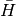and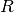to be connected?

ZERO_INTERNAL_AMPS (PSIMRCC)

PSIMRCC — Do zero the internal amplitudes, i.e., those that map reference determinants onto each other?

ZETA (CCDENSITY)

CCDENSITY — Do ?

ZETA (CCLAMBDA)

<no title>

#### Next topic

PSI Variables by Alpha